# JP4595330B2 - Image processing apparatus and method, recording medium, and program - Google Patents

## Info

Publication number
JP4595330B2
JP4595330B2 JP2004010124A JP2004010124A JP4595330B2 JP 4595330 B2 JP4595330 B2 JP 4595330B2 JP 2004010124 A JP2004010124 A JP 2004010124A JP 2004010124 A JP2004010124 A JP 2004010124A JP 4595330 B2 JP4595330 B2 JP 4595330B2
Authority
JP
Japan
Prior art keywords
luminance
image
average
smoothed
average luminance
Prior art date
Legal status (The legal status is an assumption and is not a legal conclusion. Google has not performed a legal analysis and makes no representation as to the accuracy of the status listed.)
Expired - Fee Related
Application number
JP2004010124A
Other languages
Japanese (ja)
Other versions
JP2005204195A (en
Inventor

Original Assignee
ソニー株式会社
Priority date (The priority date is an assumption and is not a legal conclusion. Google has not performed a legal analysis and makes no representation as to the accuracy of the date listed.)
Filing date
Publication date
Application filed by ソニー株式会社 filed Critical ソニー株式会社
Priority to JP2004010124A priority Critical patent/JP4595330B2/en
Publication of JP2005204195A publication Critical patent/JP2005204195A/en
Application granted granted Critical
Publication of JP4595330B2 publication Critical patent/JP4595330B2/en
Application status is Expired - Fee Related legal-status Critical
Anticipated expiration legal-status Critical

## Images

•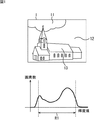•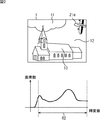••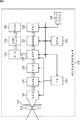•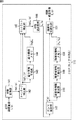••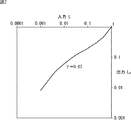•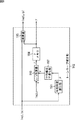•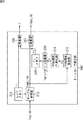•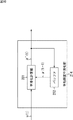•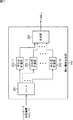•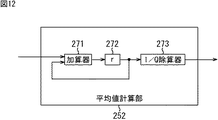•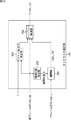•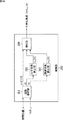•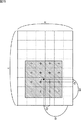••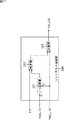•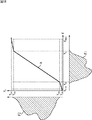••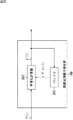•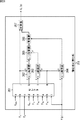•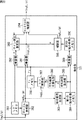•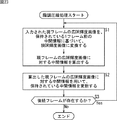•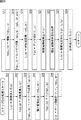••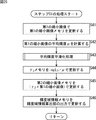•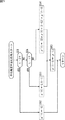•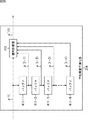•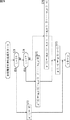•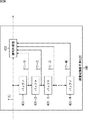•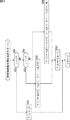••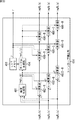•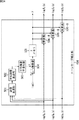•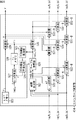••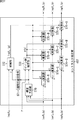•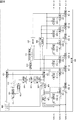•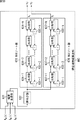•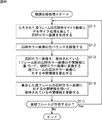•••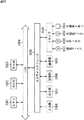## Classifications

• GPHYSICS
• G06COMPUTING; CALCULATING; COUNTING
• G06TIMAGE DATA PROCESSING OR GENERATION, IN GENERAL
• G06T5/00Image enhancement or restoration
• G06T5/40Image enhancement or restoration by the use of histogram techniques
• GPHYSICS
• G06COMPUTING; CALCULATING; COUNTING
• G06TIMAGE DATA PROCESSING OR GENERATION, IN GENERAL
• G06T5/00Image enhancement or restoration
• G06T5/007Dynamic range modification
• G06T5/009Global, i.e. based on properties of the image as a whole
• HELECTRICITY
• H04ELECTRIC COMMUNICATION TECHNIQUE
• H04NPICTORIAL COMMUNICATION, e.g. TELEVISION
• H04N5/00Details of television systems
• H04N5/14Picture signal circuitry for video frequency region
• H04N5/20Circuitry for controlling amplitude response
• H04N5/202Gamma control
• HELECTRICITY
• H04ELECTRIC COMMUNICATION TECHNIQUE
• H04NPICTORIAL COMMUNICATION, e.g. TELEVISION
• H04N5/00Details of television systems
• H04N5/222Studio circuitry; Studio devices; Studio equipment ; Cameras comprising an electronic image sensor, e.g. digital cameras, video cameras, TV cameras, video cameras, camcorders, webcams, camera modules for embedding in other devices, e.g. mobile phones, computers or vehicles
• H04N5/225Television cameras ; Cameras comprising an electronic image sensor, e.g. digital cameras, video cameras, camcorders, webcams, camera modules specially adapted for being embedded in other devices, e.g. mobile phones, computers or vehicles
• H04N5/235Circuitry or methods for compensating for variation in the brightness of the object, e.g. based on electric image signals provided by an electronic image sensor
• H04N5/243Circuitry or methods for compensating for variation in the brightness of the object, e.g. based on electric image signals provided by an electronic image sensor by influencing the picture signal, e.g. signal amplitude gain control
• HELECTRICITY
• H04ELECTRIC COMMUNICATION TECHNIQUE
• H04NPICTORIAL COMMUNICATION, e.g. TELEVISION
• H04N9/00Details of colour television systems
• H04N9/64Circuits for processing colour signals
• H04N9/68Circuits for processing colour signals for controlling the amplitude of colour signals, e.g. automatic chroma control circuits
• H04N9/69Circuits for processing colour signals for controlling the amplitude of colour signals, e.g. automatic chroma control circuits for modifying the colour signals by gamma correction

## Description

The present invention relates to an image processing apparatus and method, a recording medium, and a program, and in particular, an image processing apparatus and method, a recording medium, and a program that can realize gradation conversion corresponding to luminance fluctuation for each frame of a moving image. About.

Conventionally, the data is captured with a solid-state device such as a CCD (Charge Coupled Device), and the gradation is compressed in order to display or print on media with a lower dynamic range, and the contrast is enhanced so that it looks better. Various methods have been proposed for such processing (gradation conversion). For example, a histogram is created from the image data, and the LUT (Look Up Table) is constructed after applying appropriate transformations to the histogram based on the maximum value, minimum value, and representative value of the entire distribution. There is a technique that performs gradation conversion by using (see, for example, Patent Document 1).
JP-A-10-283470

However, since existing gradation conversion processing is considered on the premise of still images, when gradation conversion is performed with reference to both end points of the histogram of the luminance of the image, if there is a large histogram variation for each frame, At the same time, the tone conversion curve also changes, so the brightness of the entire scene changes frequently on a frame-by-frame basis, making it very unsightly.

More specifically, an example will be described. The upper part of each figure in FIGS. 1 to 3 shows three consecutive frames of a moving image, and the lower part of each figure in FIGS. 1 to 3 shows a histogram of luminance values corresponding to each frame. Yes.

The imaging data in units of frames is data having a certain dynamic range, and here, for the sake of explanation, it is simply displayed as an image 1 in which the dynamic range is compressed to 8 bits.

In addition, the brightness in each frame is highest in the airplane body part indicated by the object 21a in FIG. 2 and the object 21b in FIG. 3, and then the clouds 11 and the sky 12 in FIGS. 13 is the darkest.

It is assumed that a general contrast enhancement process is performed on such imaging data, such as assigning the upper and lower 5% points of the histogram to the highest luminance value and the lowest luminance value of the output image. In the histogram of each frame at the bottom of each of FIGS. 1 to 3, the dotted line indicates that there is a pixel within 5% above and below the width of this histogram, and this portion is assigned to the dynamic range width of the output image. .

Therefore, in FIG. 1, since the histogram range R1 is assigned to the dynamic range of the output image, high-contrast image output is expected. On the other hand, in FIG. 2, when the object 21a enters the screen, the histogram includes many high-luminance components, and the dynamic range width of the luminance assigned to the output image is widened. Therefore, since the object 21a having the highest luminance is assigned to the white level, the portion shown in the upper image of FIG. 1 is an overall dark image except for the roof 13, and the image is somewhat lost in contrast. Become.

Similarly, in the upper part of FIG. 3, the high-luminance area of the object 21b to which the object 21a has moved decreases, and the dynamic range width of the luminance allocated to the output image is narrowed. Therefore, the gradation conversion result is such that the image area other than the object 21b is slightly brighter and the contrast is increased.

When the gradation conversion result shown in the upper part of FIGS. 1 to 3 is viewed as a moving image, the brightness changes from frame to frame, and it is perceived as flickering, which is very unsightly. There was a problem.

The problems that may occur due to the gradation conversion processing have been described above. As an improvement method, for example, the gradation conversion results of the past several frames are retained, and the average of the results of the current frame is obtained. Although methods such as relieving significant fluctuations in scene brightness that occur on a unit basis are conceivable, there is a problem that the calculation cost and the amount of memory increase.

The present invention has been made in view of such a situation, and in particular, in the gradation conversion process, by smoothing the parameter controlling the luminance for each frame in the time direction, the calculation amount, and It is intended to suppress the flicker for each frame while reducing the cost of the memory capacity.

The first image processing apparatus of the present invention includes an average luminance value calculating unit that calculates an average luminance value of an image, an average luminance value of a frame to be smoothed calculated by the average luminance value calculating unit, a frame, The average luminance value of the image is time-sequentially by performing a product-sum operation using a predetermined weight on the average luminance value or smoothed average luminance value of a plurality of frames having a predetermined context in time series. Average brightness time-series smoothing means for smoothing and outputting as a smoothed average brightness value, and brightness correction for correcting the brightness of the image based on the smoothed average brightness value output by the average brightness time-series smoothing means Means , luminance area information calculating means for calculating luminance area information representing the width of the luminance distribution of the image whose brightness is corrected by the correcting means, and smoothing the luminance area information calculated by the luminance area information calculating means in time series And smoothing Luminance area information smoothing means for outputting as luminance area information, and based on the smoothed luminance area information output by the luminance area information smoothing means, the luminance of the image is adjusted in accordance with the luminance area of the display device that displays the image. Luminance region normalizing means for normalizing.

The luminance area information calculating unit is configured to calculate the luminance values at the low luminance end and the high luminance end of the luminance distribution of the image as the luminance area information.

The luminance area information smoothing means gives a predetermined weight to the luminance area information of the frame to be smoothed and the luminance area information of a plurality of frames having a predetermined chronological relationship with the frame. By performing the product-sum operation used, it is possible to smooth the luminance region information of the frame to be smoothed in time series and output the smoothed luminance region information in time series.

The luminance area information smoothing means has predetermined luminance ranges for the luminance area information of a frame to be smoothed and smoothed luminance area information of a plurality of frames having a predetermined chronological relationship with the frame. By performing the product-sum operation using the weights, it is possible to smooth the luminance region information of the frame to be smoothed in time series and output the smoothed luminance region information in time series.

The average luminance value calculation means divides the image into m × n blocks, calculates the average luminance for each block, and performs a product-sum operation using a predetermined weight on the average luminance for each block. Thus, the average luminance value of the image can be calculated.

A logarithmic conversion means for logarithmically converting the luminance value of the image and a logarithmic inverse conversion means for logarithmically inversely converting can be further provided. The average luminance value can be calculated, and the logarithmic inverse conversion means can logarithmically reverse the luminance value of the image corrected by the brightness correction means.

According to a first image processing method of the present invention, an average luminance value calculating step for calculating an average luminance value of an image, an average luminance value of a frame to be smoothed calculated by the processing of the average luminance value calculating step, The average luminance value of the image is obtained by performing a product-sum operation using a predetermined weight on the average luminance value or the smoothed average luminance value of a plurality of frames having a predetermined chronological relationship with the frame. The brightness of the image is corrected based on the average brightness time series smoothing step that is smoothed into a series and output as a smoothed average brightness value, and the smoothed average brightness value output by the process of the average brightness time series smoothing step. brightness correction step and, the luminance range information calculation step of calculating luminance range information which brightness the process of correction step represents the width of the luminance distribution of the corrected image, luminance range information calculation step of Luminance area information calculated by processing is smoothed in time series, and is output as smoothed luminance area information. Based on the smoothed luminance area information output in the luminance area information smoothing step. And a luminance range normalizing step for normalizing the luminance of the image in accordance with the luminance range of the display device that displays the image.

The first recording medium program of the present invention includes an average luminance value calculating step for calculating an average luminance value of an image, and an average luminance value of a frame to be smoothed calculated by the processing of the average luminance value calculating step. The average luminance value of the image is obtained by performing a product-sum operation using a predetermined weight on the average luminance value of a plurality of frames having a predetermined chronological relationship with the frame or the smoothed average luminance value. Based on the average brightness time series smoothing step smoothed in time series and output as a smoothed average brightness value, and the smoothed average brightness value output by the processing of the average brightness time series smoothing step, the brightness of the image is a brightness correction step of correcting, the luminance range information calculation step of calculating the luminance-region information which brightness the process of correction step represents the width of the luminance distribution of the corrected image, calculating luminance range information Luminance area information smoothed in time step, smoothed in a time series and output as smoothed luminance area information, and smoothed luminance area information output in luminance area information smoothing step And a luminance range normalizing step for normalizing the luminance of the image in accordance with the luminance range of the display device that displays the image.

The first program of the present invention includes an average luminance value calculating step for calculating an average luminance value of an image, an average luminance value of a frame to be smoothed calculated by the processing of the average luminance value calculating step, a frame, The average luminance value of the image is time-sequentially by performing a product-sum operation using a predetermined weight on the average luminance value or smoothed average luminance value of a plurality of frames having a predetermined context in time series. Brightness that corrects the brightness of the image based on the average brightness time series smoothing step that is smoothed and output as a smoothed average brightness value, and the smoothed average brightness value that is output by the processing of the average brightness time series smoothing step and the correction step, the luminance range information calculation step of brightness by the process of the correction step is to calculate the luminance range information representing the width of the luminance distribution of the corrected image, the luminance range information calculation step Luminance range information smoothed in time, smoothed in time series and output as smoothed luminance range information, and the smoothed luminance range information output in the luminance range information smoothing step processing Thus, the computer is caused to execute a luminance region normalizing step for normalizing the luminance of the image in accordance with the luminance region of the display device that displays the image.

The second image processing apparatus of the present invention includes an average luminance value calculating means for calculating an average luminance value of an image, an average luminance value of a frame to be smoothed calculated by the average luminance value calculating means , a frame, The average luminance value of the image or the smoothed average luminance value is time-series by performing a product-sum operation using a predetermined weight on the average luminance value of a plurality of frames having a predetermined context in time series. and smoothing the average luminance time series smoothing means for outputting a smoothed average luminance value, based on the output smoothed average luminance value by the average luminance time series smoothing means, the brightness of correcting the brightness of the image And a correcting means.

The average luminance value calculation means divides the image into m × n blocks, calculates the average luminance for each block, and performs a product-sum operation using a predetermined weight on the average luminance for each block. Thus, the average luminance value of the image can be calculated.

A logarithmic conversion means for logarithmically converting the luminance value of the image and a logarithmic inverse conversion means for logarithmically inversely converting can be further provided. The average luminance value can be calculated, and the logarithmic inverse conversion means can logarithmically reverse the luminance value of the image corrected by the brightness correction means.

According to a second image processing method of the present invention, an average luminance value calculating step for calculating an average luminance value of an image, an average luminance value of a frame to be smoothed calculated by the processing of the average luminance value calculating step , The average luminance value of the image or the smoothed average luminance value is obtained by performing a product-sum operation using a predetermined weight on the average luminance value of a plurality of frames having a predetermined chronological relationship with the frame in time series. Based on the average brightness time series smoothing step smoothed in time series and output as a smoothed average brightness value, and the smoothed average brightness value output in the process of average brightness time series smoothing step, the brightness of the image is And a brightness correction step for correction.

The program of the second recording medium of the present invention includes an average luminance value calculating step for calculating an average luminance value of an image, an average luminance value of a frame to be smoothed calculated by the processing of the average luminance value calculating step, and The average luminance value of the image or the smoothed average luminance value by performing a product-sum operation using a predetermined weight with respect to the average luminance value of a plurality of frames having a predetermined chronological relationship with the frame in time series the smoothed time series, and the average luminance time series smoothing step of outputting a smoothed average luminance value, based on the output smoothed average luminance value in the process of the average luminance time series smoothing step, the brightness of the image And a brightness correction step for correcting.

The second program of the present invention includes an average luminance value calculating step for calculating an average luminance value of an image, an average luminance value of a frame to be smoothed calculated by the processing of the average luminance value calculating step , a frame, The average luminance value of the image or the smoothed average luminance value is time-series by performing a product-sum operation using a predetermined weight on the average luminance value of a plurality of frames having a predetermined context in time series. and smoothing the average luminance time series smoothing step of outputting a smoothed average luminance value, based on the output smoothed average luminance value in the process of the average luminance time series smoothing step, correcting the brightness of the image A brightness correction step is executed by a computer.

In the first image processing apparatus, method, and program of the present invention, luminance region information representing the width of the luminance distribution of the image is calculated, and the calculated luminance region information is smoothed in time series, and the smoothed luminance region Based on the output smoothed luminance range information, the luminance of the image is normalized according to the luminance range of the display device that displays the image, and the average luminance value of the image is calculated and calculated. The predetermined weight is used for the average luminance value of the frame to be smoothed and the average luminance value of a plurality of frames having a predetermined chronological relationship with the frame or the smoothed average luminance value. By performing product-sum operation, the average brightness value of the image is smoothed in time series and output as a smoothed average brightness value, and the brightness of the image is corrected based on the output smoothed average brightness value. Ru is.

In the second image processing apparatus and method and program of the present invention, the average luminance value of the image is calculated, the calculated average luminance value of the frame to be smoothed, and the predetermined time-sequentially with the frame. The average brightness value of the image or the smoothed average brightness value is smoothed in time series by performing a product-sum operation using a predetermined weight on the average brightness value of multiple frames in the context. is, is output as the smoothed average luminance value, based on the output smoothed average brightness value, the brightness of the image is corrected.

The image processing apparatus of the present invention may be an independent apparatus or a block that performs image processing.

According to the present invention, it is possible to suppress flickering corresponding to luminance fluctuation for each frame of a moving image while reducing the amount of calculation in the gradation compression processing and the cost of the memory capacity.

Hereinafter, a digital video camera according to an embodiment of the present invention will be described with reference to the drawings.

FIG. 4 shows a configuration example of a digital video camera according to an embodiment of the present invention. This digital video camera 101 shoots a subject, generates a wide DR image with a wider dynamic range of pixel values than normal, records it on a predetermined storage medium, and also serves as a composition determination finder and video monitor. For a display or an external device, a wide DR image is converted into a narrow DR image having a narrower dynamic range of pixel values and output, and flickering between frames is suppressed.

The digital video camera 101 is roughly composed of an optical system, a signal processing system, a recording system, a display system, and a control system.

The optical system includes a lens 111 that collects a light image of a subject, a stop 112 that adjusts the light amount of the light image, and a CCD image that photoelectrically converts the collected light image at a predetermined frame rate to generate a wide DR image. The sensor 113 is configured. In the following, the wide DR image generated by the CCD image sensor 113 is a monochrome image composed of a luminance signal of one channel and a color image composed of a luminance signal of multiple channels (for example, three channels). Two cases will be described.

The signal processing system is a correlated double sampling circuit (CDS) 114 that reduces noise by sampling a wide DR image output from the CCD image sensor 113, and a wide DR image from which noise is removed by the correlated double sampling circuit 114. A / D converter 115 that performs AD conversion to a value having a bit width of about 14 to 16 bits, for example, and a DSP (Digital Signal Processor) that performs gradation compression processing on a wide DR image output from A / D converter 115 116.

Like a wide DR image having a bit width of 14 to 16 bits output from the A / D converter 115, an image signal with many gradations cannot be reproduced with a normal video signal such as luminance Y, color difference Cr, and Cb. However, the gradation is compressed to a range that can be reproduced by a normal video signal such as luminance Y and color differences Cr and Cb by the gradation compression processing by the DSP 116. The DSP 116 will be described in detail with reference to FIG.

The recording system of the digital video camera 101 encodes a wide DR image or a narrow DR image input from the DSP 116 and records it in the memory 122, reads out and decodes code data stored in the memory 122, and supplies it to the DSP 116. Codec (Compression / Decompression) 121 and a memory 122 made of a magnetic disk, an optical disk, a magneto-optical disk, a semiconductor, or the like for storing an encoded wide DR image or narrow DR image.

The display system includes a D / A converter 118 that DA-converts a narrow DR image supplied from the DSP 116, and an analog narrow DR image output from the D / A converter 118, and normal video signals such as luminance Y and color differences Cr and Cb. A video encoder 119 that converts the video signal into a display 120 and displays the image corresponding to the video signal. The display 120 includes an LCD (Liquid Crystal Display) that functions as a finder or a video monitor.

The control system includes a timing generator (TG) 117 that controls the operation timing of the CCD image sensor 113 to DSP 116, an input device 124 that receives various operations from the user, and a CPU (Central Processing Unit) that controls the entire digital video camera 101. ) 123.

Next, an outline of the operation of the digital video camera 101 will be described. An optical image (incident light) of a subject is incident on a CCD image sensor 113 through a lens 111 and a diaphragm 112, is photoelectrically converted by the CCD image sensor 113, and an electrical signal that becomes a pixel of the obtained wide DR image is correlated. Noise is removed by the double sampling circuit 114, digitized by the A / D converter 115, and then supplied to the DSP 116.

The DSP 116 performs gradation compression processing on the wide DR image input from the A / D converter 115, generates a narrow DR image, and outputs the narrow DR image to the D / A converter 118, the CODEC 121, or both. The narrow DR image supplied to the D / A converter 118 is DA-converted, converted into a normal video signal by the video encoder 119, and displayed on the display 120. On the other hand, the narrow DR image supplied to the CODEC 121 is encoded and recorded in the memory 122.

Next, the DSP 116 that is the main focus of the present invention will be described.

FIG. 5 shows a first configuration example of the DSP 116 corresponding to a wide DR image that is a monochrome image. Hereinafter, a monochrome wide DR image input to the DSP 116 is described as a wide DR luminance image L. Further, the pixel value (that is, the luminance value) of the wide DR luminance image is described as L (p). Here, p is a vector or coordinate indicating the pixel position in the image, such as p = (x, y). Therefore, L (p) is used separately from L representing a wide DR luminance image, assuming that it includes information on both the pixel position and the luminance value. The same applies to other images described later and their pixel values.

It is assumed that the luminance L (p) of the wide DR luminance image L is input to the DSP 116 in the raster scan order.

In the first configuration example of the DSP 116, the logarithmic conversion unit 141 performs logarithmic conversion on the input luminance L (p) and outputs the obtained logarithmic luminance logL (p) to the tone curve correction unit 142. The tone curve correction unit 142 applies a previously prepared tone curve to the input log luminance logL (p) and converts it into a direction of compressing the gradation, and the log luminance logL c (p) obtained is converted. The image is output to the reduced image generation unit 143 and the contrast correction unit 145. Further, the tone curve correction unit 142 outputs a representative value γ indicating the gradient of the applied tone curve to the contrast correction unit 145. Hereinafter, the representative value γ indicating the slope of the applied tone curve is also simply referred to as a representative value γ.

The reduced image generation unit 143 generates a reduced image logL cl based on the logarithmic luminance logL c (p) for one frame input from the tone curve correction unit 142 and stores it in the reduced image memory 144.

The contrast correction unit 145 holds the contrast weakened by the tone curve correction of the logarithmic luminance logL c (p) of the current frame input from the tone curve correction unit 142 in the representative value γ and the reduced image memory 144. Correction is performed based on the reduced image logL cl one frame before, and the obtained log luminance logL u (p) is output to the logarithmic inverse transform unit 146. The logarithmic inverse transformation unit 146 performs logarithmic inverse transformation on the logarithmic luminance logL u (p) whose contrast is corrected, and outputs the obtained normal axis luminance L u (p) to the gamma correction unit 147.

The gamma correction unit 147 performs gamma correction on the luminance L u (p) input from the logarithmic inverse conversion unit 146 in consideration of the gamma characteristic of the playback device (for example, the display 120), and obtains the obtained gamma corrected post-gamma correction The luminance Y (p) is output to the luminance region information calculation unit 148 and the luminance region normalization unit 151.

The luminance area information calculation unit 148 calculates luminance area information indicating the luminance distribution for one frame of luminance Y (p) input from the gamma correction unit 147, and calculates the luminance area information smoothing unit 149. To supply. The luminance region information smoothing unit 149 uses the luminance region information of the frame to be smoothed and the luminance region information (or smoothed luminance region information) of the frame having a predetermined relationship in the time direction, The luminance area information is smoothed and stored in the luminance area information memory 150. Here, the luminance area information is information indicating the range of the luminance distribution in one frame. For example, the luminance area information [Y represents the luminance Y d closest to the darkest and the luminance Y b closest to the brightest. d , Y b ].

The luminance region normalization unit 151 is configured to use the luminance Y (p) of the current frame input from the gamma correction unit 147 based on the luminance region information [Y d , Y b ] of the previous frame held in the luminance region information memory 150. ) So that the distribution range matches the range that can be expressed by the playback device (for example, the display 120), and the obtained luminance Y n (p) is output to the subsequent stage as the pixel value of the narrow DR image.

As described above, in the gradation compression process according to the first configuration example of the DSP 116, the reduced image logL cl is generated by the reduced image generation unit 143, and the luminance region information [Y d , Y b ] is calculated. The reduced image logL cl and the luminance area information [Y d , Y b ] are hereinafter referred to as intermediate information.

According to the DSP 116, intermediate information is calculated for each frame of the input wide DR luminance image, and the calculated intermediate information is used to process the wide DR luminance image after one frame.

In general, in order to effectively perform gradation compression, information calculated based on luminance values in the entire image or a wide range on the image is necessary, but the time lag until the information is calculated increases. This is an implementation problem. Therefore, the DSP 116 selects intermediate information that is very difficult to change in time as the information, and uses intermediate information of the previous frame for gradation compression for the current frame. By adopting such a configuration, it is possible to avoid an increase in memory usage and circuit scale even when mounted.

Next, details of each part of the first configuration example of the DSP 116 will be described with reference to the drawings.

FIG. 6 shows a first configuration example of the tone curve correction unit 142. In the first configuration example described above, the LUT memory 171 includes a lookup table (hereinafter referred to as LUT) corresponding to a monotone increasing tone curve as shown in FIG. 7 and a representative value γ indicating the slope of the tone curve. Is held in advance. Note that a function corresponding to a tone curve may be held instead of the LUT. The table reference unit 172 corrects the log luminance logL (p) to the log luminance logL c (p) based on the LUT held in the LUT memory 171.

FIG. 7 shows an example of a tone curve, where the horizontal axis represents the input luminance L (p) and the vertical axis represents the tone curve corrected luminance L c (p) normalized to [0, 1], respectively. Displayed on the logarithmic axis. As shown in this example, when a tone curve that is monotonically increasing and has a gentle inverse S-shape is applied, gradation compression does not work very strongly in the high luminance region and the low luminance region. A good color tone with little black blur is obtained. On the contrary, tone compression works strongly in the intermediate luminance range, but since the contrast correction described later is sufficiently applied to the intermediate luminance range, a good narrowness without contrast deterioration in the intermediate luminance range. A DR image is obtained.

Note that the representative value γ indicating the tone curve inclination may be obtained by, for example, obtaining the inclination of the entire luminance range and setting the average value thereof as the representative value γ. In the case of the tone curve shown in FIG. 7, the representative value γ = 0.67.

FIG. 8 shows a second configuration example of the tone curve correction unit 142. The second configuration example does not use an LUT prepared in advance as in the first configuration example, but calculates a representative value γ for each frame, and calculates the logarithmic luminance logL (p) as the logarithmic luminance logL c. The correction is made to (p). In the second configuration example, the average luminance calculation unit 191 calculates an average luminance value μ of the log luminance logL (p) for one frame and outputs the average luminance value μ to the average luminance smoothing unit 192.

The average luminance smoothing unit 192 smoothes the average luminance value μ supplied from the average luminance calculating unit 191 using the average luminance value μ of the preceding and following frames or the smoothed average luminance value μ of the preceding and following frames. And supplied to the divider 193. The divider 193 divides the predetermined constant logL T by the average luminance value μ to calculate a representative value γ. The γ memory 194 holds the representative value γ input from the divider 193. The multiplier 195 multiplies the logarithmic luminance logL (p) of the current frame by the representative value γ one frame before held in the γ memory 194 to calculate the logarithmic luminance logL c (p) after tone curve correction. To do.

Here, the predetermined constant logL T, if determined the central level of the logarithmic luminance, the average value of the logarithmic luminance logL (p) for one frame, the logarithmic luminance logL after the tone curve correction value equal logL T c (p) will be converted.

The representative value γ is calculated for each frame, but since it is determined based on the average luminance value μ of the logarithmic luminance logL (p) of each frame, it can be expected that there is not much change in the preceding and subsequent frames. . Therefore, this representative value γ is also used for tone curve correction for the current frame, as in the reduced image logL cl and luminance area information [Y d , Y b ] described above. Hereinafter, the representative value γ is also included in the intermediate information.

FIG. 9 shows a third configuration example of the tone curve correction unit 142. In other words, the third configuration example is a combination of the first configuration example and the second configuration example. In the third configuration example, the LUT memory 211 holds in advance a LUT corresponding to the tone curve as shown in FIG. 7 and a representative value γ 1 indicating the slope of the tone curve. The table reference unit 212 corrects the logarithmic luminance logL (p) to the logarithmic luminance logL c ′ (p) based on the LUT held in the LUT memory 211, and outputs it to the average luminance calculation unit 213 and the multiplier 217. .

The average luminance calculation unit 213 calculates an average luminance value μ of the log luminance logL c ′ (p) for one frame and outputs it to the average luminance smoothing unit 214. The average luminance smoothing unit 214 smoothes and divides the average luminance value supplied from the average luminance calculating unit 213 using the average luminance value of the previous and subsequent frames or the smoothed average luminance value of the previous and subsequent frames. To the vessel 215.

The divider 215 divides the predetermined constant logL T by the average luminance value μ, calculates the representative value γ 2, and stores it in the γ 2 memory 388. The multiplier 217 multiplies the logarithmic luminance logL c ′ (p) of the current frame by the representative value γ 2 of the previous frame held in the γ 2 memory 388 to obtain the log luminance logL c ( p) is calculated. Multiplier 218, representative value gamma 1, and outputs to the subsequent contrast correction section 145 a product of gamma 2 as the representative value γ (= γ 1 · γ 2 ).

Next, the configuration of the average luminance smoothing unit 214 will be described with reference to FIG. The smoothing calculation unit 231 smoothes the average brightness value μ (t) of the frame at time t to be smoothed and the smoothed average brightness value μ (t) corresponding to the frame at time (t−1) immediately before. -1) is multiplied by the respective weights for smoothing, and the result is output as a smoothed average luminance value u ′ (t) of the frame corresponding to time (t), and also to the buffer 232. Supply. The buffer 232 overwrites and stores the average luminance value u ′ (t) as the average luminance value μ (t−1), and supplies it to the smoothing calculation unit 231 as described above. By repeating this process sequentially, the smoothing calculation unit 231 sequentially smoothes the average luminance value corresponding to each frame.

Next, the configuration of the reduced image generation unit 143 will be described with reference to FIG. The sort unit 251 of the reduced image generation unit 143 belongs to the log luminance logL c (p) for one frame input from the preceding tone curve correction unit 142 when the entire image is divided into m × n blocks. It classifies according to the block and supplies it to the average value calculation units 252-1 to 252-N (= m × n). For example, what is classified into the first block is supplied to the average value calculator 252-1, and what is classified into the second block is supplied to the average value calculator 252-2. The same applies hereinafter, and those classified into the Nth block are supplied to the average value calculation unit 252-N. Hereinafter, when it is not necessary to individually distinguish the average value calculation units 252-1 to 252-N, they are simply referred to as the average value calculation unit 252.

Average value calculation section 252-i (i = 1,2, ..., N) , of one frame of the logarithmic luminance logL c (p), the logarithmic luminance logL c (p classified into the i-th block ) Is calculated and output to the combining unit 253. The synthesizing unit 253 generates an m × n pixel reduced image logL cl whose pixel value is the average value of the logarithmic luminance logL c (p) input from the average value calculating unit 252-i, and the subsequent reduced image memory 144.

FIG. 12 shows a configuration example of the average value calculation unit 252. The adder 271 of the average value calculation unit 252 adds the value held by the register (r) 272 to the logarithmic luminance logL c (p) input from the previous sorting unit 251, and the register (r) 272 holds the value. Update the value to be updated. The divider 273 divides the value finally stored in the register 272 by the number of pixels Q constituting one block, thereby obtaining Q logarithmic luminances logL c ( The average value of p) is calculated.

Next, the configuration of the contrast correction unit 145 will be described with reference to FIG. The interpolation position designation unit 291 of the contrast correction unit 145 acquires the pixel position p (hereinafter also referred to as the interpolation position p) of the logarithmic luminance logL c (p) input from the preceding tone curve correction unit 142, and performs interpolation. To the unit 292. The interpolation unit 292 calculates the pixel logL cl (p) corresponding to the interpolation position p by interpolation using the reduced image logL cl one frame before held in the reduced image memory 144 and outputs the pixel logL cl (p) to the contrast enhancement unit 294. To do.

Based on the representative value γ for the previous frame from the tone curve correction unit 142 and the log luminance logL c (p) of the current frame, the gain value setting unit 293 performs the contrast enhancement amount of the log luminance logL c (p) of the current frame. A gain value g (p) for determining the value is calculated. The contrast emphasizing unit 294 uses the logarithmic luminance logL c (p) of the current frame based on the gain value g (p) and the interpolated value logL cl (p) of the reduced image as a logarithmic luminance in which contrast other than the low-frequency component is enhanced. logL u (p) is calculated.

Next, the configuration of the interpolation unit 292 will be described with reference to FIG. The interpolation unit 292 interpolates the pixel logL cl (p) corresponding to the interpolation position p by bicubic interpolation using 4 × 4 pixels in the vicinity of the interpolation position p of the reduced image logL cl one frame before. is there.

The neighborhood selecting unit 301 receives the input of the interpolation position p, and from the reduced image logL cl of m × n pixels one frame before held in the reduced image memory 144, 4 × 4 pixels in the vicinity of the interpolation position p. Pixel values a   are acquired and output to the product-sum unit 304. Here, the notation a [i] [j] indicates that the pixel value a is i × j two-dimensional array data. In addition, the neighborhood selection unit 301 uses the horizontal coefficient calculation unit 302 or the horizontal position displacement amount dx and the vertical direction displacement amount dy between the acquired pixel value a   and the interpolation position p, respectively. The result is output to the vertical coefficient calculation unit 303.

Here, the relationship between the interpolation position p, the neighboring pixel values a  , and the positional deviation amounts dx and dy will be described with reference to FIG.

The m × n cells in FIG. 15 represent a reduced image logL cl of m × n pixels. Assuming that an interpolation position p = (px, py) is given, the position q on the reduced image logL cl corresponding to the interpolation position p is q = (qx, qy) = (px / bx−0.5, py / by-0.5). However, it is (bx, by) = (the number of horizontal pixels of the image logL c / m, the number of vertical pixels of the image logL c / n).

In order to obtain a neighboring pixel from the position q on the reduced image corresponding to the interpolation position p, the reduction is located in the range of qx−2 <x <qx + 2 and qy−2 <y <qy + 2 indicated by hatching in FIG. What is necessary is just to acquire the pixel of image logL cl . The position indicated by the 4 × 4 “+” mark in the hatched area is the pixel position to be acquired. The shift amount (dx, dy) between the neighboring pixel and the interpolation position p is a difference from the nearest pixel in the lower left direction of the interpolation position q. That is, the deviation amount (dx, dy) = (decimal part of qx, decimal part of qy).

Returning to FIG. The horizontal coefficient calculation unit 302 calculates a horizontal third-order interpolation coefficient k x  based on the horizontal shift amount dx input from the neighborhood selection unit 301. Similarly, the vertical coefficient calculation unit 303 calculates a third-order interpolation coefficient k y  in the vertical direction based on the vertical direction shift amount dy input from the neighborhood selection unit 301.

For example, the horizontal third-order interpolation coefficient k x  is calculated using the following equation (1).
... (1)
For example, the cubic interpolation coefficient k y  in the vertical direction is calculated using the following equation (2).
... (2)

In addition to the above formulas (1) and (2), the cubic interpolation coefficients k x  and k y  can be calculated by any calculation formula as long as sufficiently smooth interpolation is obtained. Can be used.

The product-sum unit 304 calculates the reduced image logL by performing a product-sum calculation of the neighboring pixel value a  , the horizontal interpolation coefficient k x , and the vertical interpolation coefficient k y . cl interpolation value L cl (p) of the interpolation position p, is calculated using the following equation (3).
... (3)

Next, the gain value setting unit 293 will be described. As described above, the gain value setting unit 293 sets the gain value g (p) that adjusts the amount of enhancement when the contrast enhancement unit 294 in the subsequent stage is enhanced except for the low frequency range. When the gain value g (p) = 1, the contrast enhancement unit 294 does not enhance or suppress the contrast. When the gain value g (p)> 1, the contrast is enhanced corresponding to the value. Further, when the gain value g (p) <1, the contrast is suppressed corresponding to the value.

An outline of gain value setting will be described. The contrast of the image has already been suppressed by tone compression by tone curve correction, but the amount of suppression depends on the slope of the tone curve. For example, if a tone curve with a small inclination is applied so as to perform strong gradation compression, the suppression of contrast is also strong. For example, if a straight line having an inclination of 1 is applied as the tone curve, the image does not change, that is, the contrast is not suppressed.

Therefore, in the gain value setting unit 293, when the representative value γ of the tone curve inclination is smaller than 1, the inverse 1 / γ of the representative value γ of the tone curve inclination is set so that the gain value is larger than 1. Adopted as a gain value.

In addition, when the input log luminance logL c (p) is close to the white level or the black level, if contrast enhancement similar to that in the intermediate luminance region is performed, clipping occurs and the image details are lost. Therefore, the gain value approaches 1 as the input log luminance logL c (p) approaches the white level or the black level.

That is, the gain value g (p) is calculated as in the following equation (4) with the reciprocal 1 / γ = g 0 of the representative value γ.
g (p) = 1 + (g 0 −1) × attn (p)
... (4)
Here, attn (p) is an attenuation coefficient, and is calculated using the following equation (5).
... (5)

In Expression (5), logL gray represents a logarithmic luminance of a moderate gray level, and logL white represents a log luminance of a white clip level (maximum white level), both of which are set in advance. It is a constant.

FIG. 16 shows a configuration example of the gain value setting unit 293. The divider 311 calculates the reciprocal 1 / γ = g 0 of the representative value γ input from the previous stage, and outputs it to the subtractor 312. The subtractor 312 calculates (g 0 −1) and outputs it to the multiplier 318.

The subtractor 313 calculates the difference (logL c (p) −logL gray ) between the logarithmic luminance logL c (p) and the logarithmic luminance logL gray of the intermediate gray level, and outputs the difference to the divider 315. The subtractor 314 calculates a difference (logL white −logL gray ) between the log luminance logL white of the white clip level and the log luminance logL gray and outputs the difference to the divider 315. The divider 315 divides the output of the subtractor 313 (logL c (p) −logL gray ) by the output of the subtractor 314 (logL white −logL gray ) and outputs the result to the absolute value calculator 316. The absolute value calculator 316 calculates the absolute value of the output of the divider 315 and outputs it to the clipper 317. When the output of the absolute value calculator 316 exceeds 1, the clipper 317 clips the value to 1, and when the output of the absolute value calculator 316 does not exceed 1, the clipper 317 retains the value as it is, and attn (p) To the multiplier 318.

Multiplier 318 multiplies the output of subtractor 312 by the output of clipper 317 and outputs the result to adder 319. The adder 319 adds 1 to the output of the multiplier 318, and outputs the calculation result to the subsequent stage as a gain value g (p).

Next, the configuration of the contrast enhancement unit 294 will be described with reference to FIG. The subtractor 321 calculates a difference (logL c (p) −logL cl (p)) between the logarithmic luminance logL c (p) and the interpolation value logL cl (p) of the reduced image, and outputs the difference to the multiplier 322. To do. The multiplier 322 calculates the product of the output of the subtractor 321 and the gain value g (p) and outputs the product to the adder 323. The adder 323 adds the interpolation value logL cl (p) of the reduced image to the output of the multiplier 322, and outputs the logarithmic luminance logL u (p) subjected to contrast correction to the subsequent stage.

Note that the interpolated value logL cl (p) of the reduced image is an interpolated value based on the reduced image of m × n pixels, and therefore has only a very low frequency component of the image logL c before the reduction. is there.

That is, the output (logL c (p) −logL cl (p)) of the subtractor 321 is obtained by subtracting only a very low frequency component from the original log luminance logL c (p). In this way, the luminance signal is separated into two components, ie, a very low frequency component and other components. Among them, the components other than the low frequency component are emphasized by multiplication by the gain value g (p), and then the adder 323 again. The logarithmic luminance logL u (p) subjected to contrast correction is synthesized by the above.

As described above, the contrast enhancement unit 294 enhances the components from the low to medium frequency range to the high frequency range except the extremely low frequency range with the same gain value g (p). Therefore, the logarithmic luminance logL u (p) subjected to the contrast correction is noticeable when only the high frequency region is emphasized, and the local overshoot of the edge portion does not occur, and the contrast is enhanced naturally. An image can be obtained.

Next, the luminance area information calculation unit 148 and the luminance area normalization unit 151 will be described.

First, an outline of the luminance range normalization process will be described. The purpose of the tone compression processing by the DSP 116 is to convert a wide DR luminance image into a narrow DR image suitable for the dynamic range of the playback device such as the display 120. For this purpose, a tone curve that matches the dynamic range of the playback device. Is prepared in advance in the tone curve correction unit 142. As a result, it is possible to appropriately perform gradation compression on a large number of captured wide DR luminance images.

However, depending on the subject to be photographed, the dynamic range of incident light may not be so large from the beginning, so when gradation compression processing is performed on the image, gradation compression is performed more than necessary, and the playback device The brightness may be pushed into a narrower range than the reproducible dynamic range.

In order to suppress this, the luminance region normalization unit 151 converts the dynamic range of the luminance signal Y (p) after the gamma correction into a dynamic range that can be reproduced by the reproduction device, as the final stage of the gradation compression processing. The luminance signal Y (p) after gamma correction is normalized so as to match.

FIG. 18 shows how the luminance range normalization unit 151 performs luminance range normalization processing. In the line graph of FIG. 8, the horizontal axis indicates the luminance Y after gamma correction before luminance region normalization, the vertical axis indicates the luminance Y n after luminance region normalization, and the gradation conversion curve α the Y shows the conversion table used when converting Y n.

A method for obtaining the gradation conversion curve α will be described. A hatched figure F1 shown below the line graph is an example of a histogram of the luminance image Y before luminance region normalization. In this example, gradation compression is performed to a dynamic range narrower than the dynamic range from the lowest luminance Y min that can be generated by the digital video camera 101 to the highest luminance Y max before the luminance region normalization after the gamma correction is performed. The obtained luminance image is obtained.

If output to the playback device in this dynamic range, the playback device's reproducible dynamic range will not be used effectively, so the brightness distribution of the brightness image Y before normalization of the brightness range will cover the entire dynamic range of the playback device. Normalization is performed so that it is distributed over.

For this purpose, first, a range [Y d , Y b ] in which a histogram shape such as the figure F1 of the luminance image Y before luminance region normalization is distributed is calculated as luminance region information of the luminance image Y before luminance region normalization. . Then, luminances Y na and Y ns that are slightly inside the upper and lower ends of the luminance range [Y nb , Y nc ] of the playback device are set, and the horizontal axis luminances {Y min , Y d , Y b , Y max } are The gradation conversion curve α is determined so as to correspond to the luminance {Y nb , Y na , Y ns , Y nc } on the vertical axis.

If gradation conversion is performed using the gradation conversion curve α, a luminance image Y n having a histogram shape like the hatched figure F2 shown on the left side of the line graph can be obtained.

Note that the luminance range [Y d , Y b ] before normalization of the luminance range is mapped to a luminance range [Y na , Y ns ] slightly narrower than the luminance range [Y nb , Y nc ] of the playback device, The gradation conversion curve α is determined in order to prevent abrupt luminance clipping in the vicinity of the luminances Y nb and Y nc from appearing on the image.

Here, it is assumed that the luminances Y na and Y ns are set to appropriate values in advance based on the luminances Y nb and Y nc .

Note that the luminance region information [Y d , Y b ] before the luminance region normalization is acquired by the luminance region information calculation unit 148, and the determination of the gradation conversion curve α and the calculation of the luminance Y n (p) are performed in the luminance region. The normalization unit 151 executes. Further, the luminance area information before the luminance area normalization is smoothed by a luminance area information smoothing unit 148 described later.

Next, the configuration of the luminance area information calculation unit 148 will be described with reference to FIG. In the luminance area information calculation unit 148, the thinning unit 341 selects the luminance Y (p) input from the gamma correction unit 147 based on the pixel position p. That is, only the luminance of the pixel at the preset pixel position is supplied to the subsequent MIN sorting unit 342 and MAX sorting unit 345.

In the MIN sort unit 342, k combinations of the comparison unit 343 and the register 344 are arranged in series, and the input luminance Y (p) is held in the registers 344-1 to 344-k in ascending order. Yes.

For example, the comparison unit 343-1 compares the luminance Y (p) from the thinning-out unit 341 with the value of the register 344-1, and the luminance Y (p) from the thinning-out unit 341 is smaller than the value of the register 344-1. In this case, the value of the register 344-1 is updated using the luminance Y (p) from the thinning unit 341. On the contrary, when the luminance Y (p) from the thinning-out unit 341 is not smaller than the value of the register 344-1, the luminance Y (p) from the thinning-out unit 341 is supplied to the comparison unit 343-2 at the subsequent stage.

The comparison unit 343-2 compares the luminance Y (p) from the comparison unit 343-1 with the value of the register 344-2, and the luminance Y (p) from the comparison unit 343-1 is based on the value of the register 344-2. Is smaller, the value of the register 344-2 is updated using the luminance Y (p) from the comparison unit 343-1. On the other hand, when the luminance Y (p) from the comparison unit 343-1 is not smaller than the value of the register 344-2, the luminance Y (p) from the comparison unit 343-1 is supplied to the comparison unit 343-3 at the subsequent stage. Is done.

The same applies in the following comparison unit 343-3, one frame of the luminance Y (p) in the step input, the minimum value Y min of the luminance Y (p) is held in the register 344-1, the register 344- 2 to 344-k, will be the luminance Y (p) is held in the ascending order, held in the register 344-k luminance Y (p) is output to the subsequent stage as the luminance Y d of the luminance range information .

The MAX sort unit 345 has k combinations of the comparison unit 346 and the register 347 arranged in series, and holds the input luminance Y (p) in the registers 347-1 to 347-k in descending order. Yes.

For example, the comparison unit 346-1 compares the luminance Y (p) from the thinning unit 341 and the value of the register 347-1, and the luminance Y (p) from the thinning unit 341 is larger than the value of the register 344-1. In this case, the value of the register 347-1 is updated using the luminance Y (p) from the thinning unit 341. On the other hand, when the luminance Y (p) from the thinning-out unit 341 is not larger than the value of the register 347-1, the luminance Y (p) from the thinning-out unit 341 is supplied to the comparison unit 346-2 at the subsequent stage.

The comparison unit 346-2 compares the luminance Y (p) from the comparison unit 346-1 with the value of the register 347-2, and the luminance Y (p) from the comparison unit 346-1 is based on the value of the register 347-2. Is also larger, the value of the register 347-2 is updated using the luminance Y (p) from the comparison unit 346-1. On the other hand, when the luminance Y (p) from the comparison unit 346-1 is not greater than the value of the register 347-2, the luminance Y (p) from the comparison unit 346-1 is supplied to the comparison unit 346-3 at the subsequent stage. Is done.

The same applies to the comparison unit 346-3 and later, and when the luminance Y (p) for one frame is input, the maximum value Y max of the luminance Y (p) is held in the register 347-1, and the register 347- 2 to 347-k, will be retained in order luminance Y (p) is large, held in the register 347-k luminance Y (p) is output to the subsequent stage as the luminance Y b of the luminance range information .

Note that the luminance Y (p) input to the MIN sorting unit 342 and the MAX sorting unit 345 is thinned out by the thinning unit 341. Therefore, the thinning interval and the number of stages of the MIN sorting unit 342 and the MAX sorting unit 345 are determined. If k is appropriately adjusted, it is possible to obtain luminances Y d and Y b corresponding to, for example, 1% of the upper limit and the lower limit of all pixels in one frame, for example.

Next, the configuration of the luminance area information smoothing unit 149 will be described with reference to FIG. In the description of the luminance area smoothing process, the luminances Y d and Y b at time t that define the luminance area information are unified and expressed as Y (t). Therefore, the brightness area smoothing unit 149 performs the smoothing process on the brightness Y d and Y b of the pixel p defining the brightness area information at the time t in the same manner, and then performs the brightness area information memory in the subsequent stage. It is assumed that the brightness area information smoothed to 150 is output.

The smoothing calculation unit 351 includes the luminance region information Y (t) of the frame at time t to be smoothed and the luminance region information Y (t−1) corresponding to the frame at the previous time (t−1). The luminance area information Y (t) is smoothed by obtaining the sum multiplied by the respective weights, and is output as the luminance area information Y ′ (t) of the frame corresponding to the time (t) and supplied to the buffer 352. To do. The buffer 352 overwrites and stores the luminance area information Y ′ (t) as the luminance area information Y (P, t−1), and supplies it to the smoothing calculation unit 351 as described above. By repeating this process sequentially, the smoothing calculation unit 351 sequentially smoothes the average luminance value corresponding to each frame.

FIG. 21 illustrates a configuration example of the luminance range normalization unit 151. As described above, the luminance region normalization unit 151 determines the gradation conversion curve α, and uses the gradation conversion curve α to convert the luminance Y (p) after gamma correction to the luminance Y n ( p).

As shown in FIG. 18, since the gradation conversion curve α is composed of five line segments, the luminance range normalization unit 151 determines in which line segment the input luminance Y (p) is. The luminance Y n (p) after normalizing the luminance range is determined by applying one of the five line segments constituting the gradation conversion curve α to the input luminance Y (p). Convert to

The selector 361 of the luminance range normalization unit 151 is configured to input the luminances Y max , Y b , Y d , Y min , Y inputted to the input terminals a to h based on the luminance Y (p) inputted to the input terminal i. Four luminances among nc , Y ns , Y na , and Y nb are output from the output terminals j to m. This correspondence is as follows.

The subtractor 362 calculates the difference between the output at the output terminal k and the output at the output terminal j, and outputs the difference to the divider 365. The subtractor 363 calculates the difference between the output of the output terminal l and the output of the subtracter 364 and outputs the difference to the divider 365. The subtractor 364 calculates the difference between the luminance Y (p) and the output from the output terminal m, and outputs the difference to the multiplier 366. The divider 365 calculates the ratio between the output of the subtractor 362 and the output of the subtracter 363 and outputs the result to the multiplier 366. Multiplier 366 calculates the product of the output of divider 365 and the output of subtractor 364 and outputs the result to adder 367. The adder 367 adds the output of the output terminal j and the output of the multiplier 366 and outputs the result.

The output Y n (p) of the adder 367 is as shown in the following equation (6) indicating the line segment of the gradation conversion curve α determined based on the luminance Y (p) after gamma correction.
... (6)

By the way, if attention is paid to the fact that the average luminance calculation unit 213 of the tone curve correction unit 142 shown in FIG. 9 and the average value calculation unit 252 of the reduced image generation unit 143 shown in FIG. It is possible to reduce the amount of calculation with a simpler circuit configuration. Specifically, the tone curve correction unit 142, the reduced image generation unit 143, the reduced image memory 144, and the contrast correction unit 145 shown in FIG. 5 may be combined to provide a composite unit 371 as shown in FIG.

The composite unit 371 can replace the tone curve correction unit 142, the reduced image generation unit 143, the reduced image memory 144, and the contrast correction unit 145 shown in FIG.

The LUT memory 381 of the composite unit 371 holds in advance a LUT corresponding to the tone curve as shown in FIG. 7 and a representative value γ 1 indicating the slope of the tone curve. The table reference unit 382 corrects the logarithmic luminance logL (p) input from the upper stage to the logarithmic luminance logL c ′ (p) based on the LUT held in the LUT memory 381, and reduces the reduced image generation unit 383 and the multiplication. Output to the unit 392.

The reduced image generation unit 383 divides the log luminance image logL c ′ into m × n blocks, calculates an average value of log luminances log L c ′ (p) of pixels belonging to each block, and obtains m × n pixels. The first reduced image is generated and held in the first reduced image memory 384.

The average luminance calculation unit 385 calculates the average luminance value μ of the pixel values of the first reduced image one frame before held in the first reduced image memory 384 and outputs the average luminance value μ to the average luminance smoothing unit 386. .

The average luminance smoothing unit 386 smoothes and divides the average luminance value supplied from the average luminance calculating unit 385 using the average luminance value of the preceding and following frames or the smoothed average luminance value of the preceding and following frames. To the vessel 387.

The divider 387 divides the predetermined constant logL T by the smoothed average luminance value μ, calculates the representative value γ 2, and stores it in the γ 2 memory 388. The multiplier 389 multiplies each pixel of the first reduced image held in the first reduced image memory 384 by the representative value γ 2 held in the γ 2 memory 388 to obtain the second reduced image. logL cl is generated and stored in the second reduced image memory 390.

The multiplier 391 multiplies the logarithmic luminance logL c ′ (p) of the current frame input from the table reference unit 382 by the representative value γ 2 of the previous frame held in the γ 2 memory 388 to obtain a tone curve. The logarithmic luminance logL c (p) after correction is calculated. The multiplier 392 outputs the representative value gamma 1, the product of gamma 2 representative value γ (= γ 1 · γ 2 ) the gain value setting section 393 as.

Gain value setting section 393, a representative value γ for the previous frame input from the multiplier 392, based on the logarithmic luminance logL c of the current frame input (p) from the multiplier 391, the logarithmic luminance logL c of the current frame A gain value g (p) that determines the contrast enhancement amount of (p) is calculated.

The interpolation position specifying unit 394 acquires the pixel position p (hereinafter also referred to as the interpolation position p) of the logarithmic luminance logL c (p) of the current frame input from the multiplier 391 and outputs the pixel position p to the interpolation unit 174. The interpolation unit 174 calculates the pixel logL cl (p) corresponding to the interpolation position p by interpolation using the second reduced image logL cl one frame before held in the second reduced image memory 390. The result is output to the contrast enhancement unit 394.

The contrast enhancement unit 394 uses the gain value g (p) and the reduced image interpolated value logL cl (p) for the logarithmic luminance logL c (p) of the current frame input from the multiplier 391, except for the low frequency component. Logarithmic luminance logL u (p) with enhanced contrast is calculated.

If this composite unit 371 is used, the average luminance calculation unit 385 calculates the average value of m × n pixels of the first reduced image, so that the average of the pixels of the log luminance image logL c of the original image size is calculated. Compared with the average luminance calculation unit 213 in FIG. 9 that calculates a value, the amount of calculation can be reduced. Therefore, the delay time resulting from the calculation can be shortened.

Next, comprehensive tone compression processing according to the first configuration example of the DSP 116 to which the composite unit 371 shown in FIG. 22 is applied will be described with reference to the flowchart of FIG.

In step S 1, the DSP 116 calculates the intermediate information (second reduced image logL c (p ), The representative value γ, and luminance area information [Y d , Y b ]), are converted into a narrow DR luminance image Y n . Further, the DSP 116 calculates intermediate information for the wide DR luminance image L of the current frame.

In step S2, the DSP 116 uses the calculated intermediate information for the wide DR luminance image L of the current frame to update the intermediate information for the wide DR luminance image of the previous frame.

In step S3, the DSP 116 determines whether or not there is a subsequent frame in the input wide DR luminance image of the current frame. If it is determined that there is a subsequent frame, the DSP 116 returns to step S1, and thereafter Repeat the process. On the contrary, if it is determined that there is no subsequent frame, the gradation compression process is terminated.

Details of the processing in units of pixels in step S1 will be described with reference to the flowchart of FIG. The processing of each step described below is executed for the target pixel (pixel position p) input in the raster scan order.

In step S <b> 11, the luminance L (p) of the target pixel (pixel position p) is input to the DSP 116. In step S <b> 12, the logarithmic conversion unit 141 performs logarithmic conversion on the input luminance L (p) and outputs the obtained logarithmic luminance logL (p) to the composite unit 371. In step S13, the table reference unit 382 of the multifunction unit 371, the logarithmic luminance logL input from logarithmic conversion section 141 (p), LUT logarithmic luminance logL based on the LUT held in the memory 381 c '(p) And output to the reduced image generation unit 383 and the multiplier 392. At the same time, the LUT memory 381 outputs the representative value γ 1 of the tone curve slope to the multiplier 392. Multiplier 392, the output of the gain value setting section 393 a product of the calculated gamma 2 on the basis of one frame before the first reduced image held by the representative value gamma 1 and gamma 2 memory 388 as a representative value gamma To do.

In step S14, the reduced image generation unit 383 generates a first reduced image based on the logarithmic luminance logLc ′ (p) for one frame after the tone curve correction. Here, the representative value γ 2 is calculated based on the generated first reduced image. In addition, the generated first reduced image is multiplied by the calculated representative value γ 2 to generate the second reduced image logL cl .

In step S15, the multiplier 391 multiplies the logarithmic luminance logL c ′ (p) of the current frame input from the table reference unit 382 by the representative value γ 2 of the previous frame held in the γ 2 memory 388. Thus, the logarithmic luminance logL c (p) after tone curve correction is calculated.

In step S16, the gain value setting unit 393 determines the current frame based on the representative value γ for the previous frame input from the multiplier 392 and the logarithmic luminance logL c (p) of the current frame input from the multiplier 391. A gain value g (p) that determines the contrast enhancement amount of the logarithmic luminance logL c (p) is calculated.

In step S <b> 17, the interpolation unit 174 interpolates the pixel logL cl (p) corresponding to the interpolation position p, using the second reduced image logL cl one frame before held in the second reduced image memory 390. Is calculated and output to the contrast enhancement unit 394. In step S18, the contrast emphasizing unit 394, based on the interpolation value logL cl (p) and the gain value g (p) of the second reduced image, the low frequency band component of the log luminance logL c (p) after tone curve correction. The log luminance logL u (p) corrected as a result is output to the logarithmic inverse transform unit 146 at the subsequent stage.

In step S <b> 19, the logarithmic inverse conversion unit 146 converts the contrast-corrected logarithmic luminance logL u (P) into the normal axis luminance L u (P) and outputs it to the gamma correction unit 147. In step S20, the gamma correction unit 147 performs predetermined gamma correction, and outputs the obtained luminance Y (p) to the luminance region information calculation unit 148 and the luminance region normalization unit 151.

In step S21, the luminance area information calculation unit 148 generates luminance area information [Y d , Y b ] based on the luminance Y (p) for one frame.

In step S < b > 22, the luminance area information smoothing unit 149 performs luminance area information smoothing processing, smoothes the luminance area information [Y d , Y b ], and stores the information in the luminance area information memory 150. The luminance area information smoothing process will be described later with reference to the flowchart of FIG.

In step S < b > 23, the luminance region normalization unit 151 is input from the gamma correction unit 147 based on the luminance region information [Y d , Y b ] that has been smoothed by one frame held in the luminance region information memory 150. The obtained luminance Y (p) is normalized to calculate the luminance Y n (p). In step S24, the luminance region normalization unit 151 outputs the luminance Y n (p) as the pixel value of the narrow DR luminance image subjected to gradation compression.

Here, with reference to the flowchart of FIG. 25, the brightness | luminance area information smoothing process which is a process of step S23 in the flowchart of FIG. 24 is demonstrated. In the following description of the luminance area information smoothing process, the luminance Y d and Y b of the luminance area information [Y d , Y b ] at time t are described as Y (t).

In step S31, the smoothing calculation unit 351 of the luminance range information smoothing unit 149 determines whether or not the input frame time t is 0. That is, it is determined whether it is the first frame. If it is determined in step S31 that the time t = 0, in step S32, the smoothing calculation unit 351 uses the predetermined constant V1 as the smoothed luminance region information Y ′ (t) corresponding to the time t. The data is output and supplied to the buffer 352.

In step S33, the buffer 352 overwrites and stores the smoothed luminance area information Y ′ (t) as smoothed luminance area information Y ′ (t−1) one frame before.

In step S31, when it is determined that the time t is not 0, that is, it is determined that the luminance area information Y (t) corresponding to any frame, not the first frame, has been supplied before that, In step S34, the smoothing calculation unit 351 determines whether or not the time t = 1, that is, whether or not the luminance area information Y (t) (t = 1) of the second frame. If it is the eye luminance range information Y (t) (t = 1), the processing proceeds to step S35.

In step S35, the smoothing calculation unit 351 has the luminance area information Y (t-1) of the immediately preceding frame stored in the buffer 352 (the luminance area information Y (t) (t = 0) since it is the second frame). Is output as a result of smoothing the luminance area information Y (t), and the process proceeds to step S33.

If it is determined in step S34 that the time t is not 1, that is, the third frame or later, the process proceeds to step S36.

In step S <b> 36, the smoothing calculation unit 351 determines the luminance region information Y (t) of the frame that has been input, and the smoothed luminance region information Y ′ (t−1) of the previous frame stored in the buffer 352. ), C1 × Y (t) + (1−C1) × Y ′ (t−1) is calculated and output as smoothed luminance area information Y ′ (t) of the currently input frame. The process proceeds to step S33. Here, the weighting coefficient C1 is an arbitrary value that is larger than 0 and smaller than 1.

By repeating the above process, the luminance area information is sequentially smoothed in consideration of the smoothed luminance area information of the immediately preceding frame, so gradation conversion corresponding to the luminance fluctuation for each frame of the moving image Can be realized. For example, in this case, the weighting factor C1 is smoothed in a state where the value of the luminance area information of the current frame is considered as the value closer to 1, and conversely, the value closer to 0 becomes the immediately preceding value. Smoothing is performed in a state where the luminance range information of the frame is taken into consideration. Further, in the present example, the example of smoothing the luminance area information based on the luminance area information smoothed immediately before and the luminance area information that has just been input has been described. Smoothing may be performed using the brightness area information smoothed in step S1 and the brightness area information that has been input.

Next, details of the processing in step S2 in FIG. 23 will be described with reference to the flowchart in FIG. In step S41, the reduced image generation unit 383 holds the first reduced image memory 384 using the first reduced image generated based on the logarithmic luminance logLc ′ (p) for one frame after the tone curve correction. The first reduced image being updated is updated.

In step S <b> 42, the average luminance calculation unit 385 calculates an average luminance value μ based on the pixel value of each pixel in the first reduced image memory 384 and supplies the average luminance value μ to the average luminance smoothing unit 386.

In step S <b> 43, the average brightness smoothing unit 386 performs an average brightness smoothing process, smoothes the supplied average brightness value μ, and supplies the smoothed average brightness value μ to the divider 387. The average brightness smoothing process will be described later in detail with reference to the flowchart of FIG.

In step S44, the divider 387 calculates the representative value γ 2 by dividing the predetermined constant logL T by the smoothed average luminance value μ input from the average luminance calculating unit 385, and calculates the representative value thus calculated. using gamma 2, and updates the representative value gamma 2 held by the gamma 2 memory 388.

In step S45, the multiplier 389 adds the γ 2 memory updated in the process of step S44 to each pixel of the first reduced image held in the first reduced image memory 384 updated in the process of step S41. 388 by multiplying the representative value gamma 2 held in, to generate a second reduced image logL cl, and updates the second reduced image logL cl held by the first reduced image memory 390.

In step S46, the luminance area information calculation unit 148 is held in the luminance area information memory 150 using the luminance area information [Y d , Y b ] calculated based on the luminance Y (p) for one frame. The brightness area information [Y d , Y b ] of the previous frame is updated.

Here, with reference to the flowchart of FIG. 27, the average brightness | luminance smoothing process which is a process of step S43 in the flowchart of FIG. 26 is demonstrated. In the following description, the average luminance μ at time t is assumed to be μ (t).

In step S51, the smoothing calculation unit 231 of the average luminance smoothing unit 214 determines whether or not the input frame time t is zero. That is, it is determined whether it is the first frame. When it is determined in step S51 that time = 0, in step S52, the smoothing calculation unit 231 outputs a predetermined constant V2 as a smoothed average luminance μ ′ (t) corresponding to time t. At the same time, it is supplied to the buffer 232.

In step S53, the buffer 232 overwrites and stores the smoothed average luminance value μ ′ (t) as the smoothed average luminance value μ ′ (t−1) of the previous frame.

In step S51, when it is determined that the time t is not 0, that is, it is determined that the average luminance value μ (t) corresponding to any one of the frames is supplied before the first frame, In step S54, the smoothing calculation unit 231 determines whether or not time t = 1, that is, whether or not the average luminance value μ (1) of the second frame, for example, the average luminance value of the second frame. If μ (1), the process proceeds to step S55.

In step S <b> 55, the smoothing calculation unit 231 uses the average luminance value μ (t−1) of the immediately previous frame stored in the buffer 232 (the average luminance value μ (0) because it is the second frame) as the average luminance value. The result of smoothing μ (t) is output, and the process proceeds to step S53.

If it is determined in step S54 that the time t is not 1, that is, the third frame or later, the process proceeds to step S56.

In step S <b> 56, the smoothing calculation unit 231 determines the average luminance value μ (t) of the currently input frame and the smoothed average luminance value μ ′ (t−1) of the immediately previous frame stored in the buffer 232. ), C2 × μ (t) + (1−C2) × μ ′ (t−1) is calculated and output as the smoothed average luminance value μ ′ (t) of the frame that has just been input. The process proceeds to step S53. Here, the weighting coefficient C2 is an arbitrary value that is larger than 0 and smaller than 1.

By repeating the above processing, the average luminance value is sequentially smoothed in consideration of the smoothed average luminance value of the immediately preceding frame, so gradation conversion corresponding to the luminance fluctuation for each frame of the moving image Can be realized. For example, in the present case, the weight coefficient C2 is smoothed in a state where the value of the average luminance value of the current frame is considered as the value is closer to 1, and conversely, the value closer to 0 is the immediately preceding value. Smoothing is performed with the average luminance value of the frame taken into account. Further, in the present example, the example of smoothing the average luminance value μ based on the average luminance value smoothed immediately before and the average luminance value input now has been described. Smoothing may be performed using the average luminance value smoothed at the timing and the input average luminance value.

In the above processing, the method of smoothing the average luminance value of the next frame using the processing result before processing has been described, but this processing is a kind of so-called infinite impulse response (IIR) filter. Depending on the coefficients used in the calculation, the result may be unstable.

Therefore, as in the case of a finite impulse response (FIR) type filter, smoothing may be performed by using an average luminance value corresponding to a certain past input frame so as to stabilize the result.

FIG. 28 shows the configuration of the FIR type average luminance smoothing unit 214.

The buffers 411-1 to 411-N are basically the same as each other. When the average luminance value μ (t) is supplied at time t, the buffer 411-1 multiplies the average luminance value μ (t−1) stored at time (t−1) which is the previous timing. While supplying to the calculation part 412, it supplies to the buffer 411-2. Similarly, the buffer 411-n stores the average luminance value μ (t) supplied from the buffer 411- (n−1) and the average luminance value μ stored immediately before in the buffer 411- (n + 1). (T-1) is supplied to the product-sum calculation unit 412.

As a result, the buffers 411-1 to 411-N respectively calculate the average luminance values μ (t−1) to μ (t−N) up to the time t−N from the current time t, respectively. To supply.

The product-sum calculation unit 412 performs a product-sum operation using a predetermined weighting factor based on the input average luminance values μ (t) to μ (t−N), thereby obtaining an average luminance value μ (t). Is smoothed and output as a smoothed average luminance value μ ′ (t).

Next, the average luminance smoothing process by the average luminance smoothing unit 214 of FIG. 28 will be described with reference to the flowchart of FIG.

In step S71, the product-sum calculation unit 412 of the average luminance smoothing unit 214 in FIG. 28 determines whether or not the input frame time t is zero. That is, it is determined whether it is the first frame. In step S71, when it is determined that time = 0, in step S72, the product-sum calculation unit 412 outputs a predetermined constant V2 as a smoothed average luminance value μ ′ (t) corresponding to time t. At the same time, the buffer 411-1 stores the input average luminance value μ (t) (= average luminance value μ (0)).

In step S73, the buffer 411-n (n = 1, 2, 3... N) supplies the average luminance value μ (t−n) stored in the buffer 411- (n + 1), and the buffer 411- The average luminance value μ (t) supplied from (n−1) is overwritten and stored as the average luminance value μ (t−n).

In step S71, when it is determined that the time t is not 0, that is, it is determined that the average luminance value μ (t) corresponding to any one of the frames is supplied before the first frame, In step S74, the product-sum calculation unit 412 determines whether time t ≦ N, that is, whether the average luminance value μ (t) is not stored in all of the buffers 411-1 to 411-N. For example, if the average luminance value is not stored in all, the process proceeds to step S75.

In step S75, the product-sum calculation unit 412 calculates the average of the frame immediately before the buffer 411-1 to 411-t in which the average luminance value μ is already stored and the current frame among the buffers 411-1 to 411-N. the luminance value mu (t) to mu (0), respectively multiplied by the weighting factor a 0 to a t, and outputs the average luminance value mu (t) average luminance value mu of the smoothed '(t), the process Advances to step S73. More particularly, the product sum calculation section 412, a 0 μ (t) + a 1 μ (t-1) + a 2 μ (t-2) + ··· + a t calculated mu and (0), the smoothed The average luminance value μ ′ (t) is output. Here, the weighting factor, a 0 + a 1 + a 2 + ··· + a t = 1 becomes an arbitrary value respectively.

If it is determined in step S74 that the time t ≦ N is not satisfied, that is, it is after the Nth frame, the processing proceeds to step S76.

In step S76, the product-sum calculation unit 412 adds weight coefficients a 0 to a to the average luminance values μ (t) to μ (0) of the frames stored in the buffers 411-1 to 411-N and the current frame, respectively. Multiplying a N and outputting the average luminance value μ (t) as a smoothed average luminance value μ ′ (t), the process proceeds to step S73. More specifically, the product-sum calculation unit 412 calculates a 0 μ (t) + a 1 μ (t−1) + a 2 μ (t−2) +... + An μ (t−n) The smoothed average luminance value μ ′ (t) is output. Here, the weighting coefficients are a 0 + a 1 + a 2 +... + An = 1, each being an arbitrary value.

By repeating the above processing, the average luminance value is sequentially smoothed in consideration of the smoothed average luminance value of the immediately preceding frame, so gradation conversion corresponding to the luminance fluctuation for each frame of the moving image Can be realized. In addition, this process uses an average luminance value corresponding to a certain past input frame, as in a finite impulse response (FIR) type filter, and smoothes the result, so that the result is stabilized. be able to.

In the above, the FIR type average luminance smoothing process has been described. However, the luminance area information smoothing process may be an FIR type.

FIG. 30 shows the configuration of the FIR type luminance area information smoothing unit 149.

The buffers 431-1 to 431-M are basically the same as each other. When the luminance area information Y (t) is supplied at the time t, the buffer 411-1 multiplies the luminance area information Y (t-1) stored at the previous time (t-1). While supplying to the calculation part 432, it supplies to the buffer 431-2. Similarly, the buffer 431-n stores the luminance region information Y (t) supplied from the buffer 431- (n-1), and also stores the luminance region information Y stored immediately before in the buffer 431- (n + 1). (T-1) is supplied and supplied to the product-sum calculation unit 432.

As a result, the buffers 431-1 to 431 -M receive the luminance area information Y (t−1) to Y (t−N) up to the time t−N from the current time t, respectively. To supply.

The product-sum calculation unit 432 performs a product-sum operation using a predetermined weighting factor based on the input luminance region information Y (t) to Y (t−M), thereby obtaining luminance region information Y (t). Is smoothed and output as smoothed luminance region information Y ′ (t).

Next, the average luminance smoothing process by the luminance region information smoothing unit 149 of FIG. 30 will be described with reference to the flowchart of FIG.

In step S91, the product-sum calculation unit 432 of the luminance range information smoothing unit 149 in FIG. 30 determines whether or not the input frame time t is zero. That is, it is determined whether it is the first frame. If it is determined in step S91 that time = 0, in step S92, the product-sum calculation unit 432 outputs a predetermined constant V1 as smoothed luminance region information Y ′ (t) corresponding to time t. At the same time, the buffer 431-1 stores the input luminance area information Y (t) (= luminance area information Y (t) (t = 0)).

In step S93, the buffer 431-n (n = 1, 2, 3... M) supplies the luminance area information Y (t−1) stored in the buffer 431- (n + 1), and the buffer 431- The luminance area information Y (t) supplied from (n-1) is overwritten and stored as luminance area information Y (t-1).

In step S91, when it is determined that the time t = 0 is not 0, that is, it is determined that the luminance area information Y (t) corresponding to any one of the frames is supplied before the first frame, In step S94, the product-sum calculation unit 432 determines whether time t ≦ M, that is, whether or not the luminance region information Y (t) is stored in all of the buffers 431-1 to 431-M. For example, if the luminance area information Y is not stored in all, the process proceeds to step S95.

In step S95, the product-sum calculation unit 432 compares the luminance of the frame immediately before and the current frame of the buffers 431-1 to 431-t in which the luminance area information Y is already stored among the buffers 431-1 to 431-M. in-band information Y (t) to mu (0), respectively is multiplied by a weighting factor b 0 to b t, and outputs the luminance range information Y (t) as the luminance range information obtained by smoothing Y '(t), the process Advances to step S93. More specifically, the product-sum calculation unit 432 calculates b 0 Y (t) + b 1 Y (t−1) + b 2 Y (t−2) +... + B t Y (0) and performs smoothing. Is output as the luminance region information Y ′ (t). Here, the weighting coefficients are b 0 + b 1 + b 2 +... + B t = 1, which are arbitrary values.

If it is determined in step S94 that the time t ≦ M is not satisfied, that is, it is after the Mth frame, the process proceeds to step S96.

In step S96, the product-sum calculation unit 432 assigns weighting factors b 0 to b 0 to the luminance region information Y (t) to Y (0) of the current frame and the frames stored in the buffers 431-1 to 431-M, respectively. Multiply by b N and output the brightness area information Y (t) as smoothed brightness area information Y ′ (t), and the process proceeds to step S93. More specifically, the product-sum calculation unit 432 calculates b 0 Y (t) + b 1 Y (t−1) + b 2 Y (t−2) +... + B M Y (t−M), Output as smoothed luminance area information Y ′ (t). Here, the weighting coefficients are b 0 + b 1 + b 2 +... + B M = 1 and are arbitrary values.

By repeating the above process, the luminance area information is sequentially smoothed in consideration of the smoothed luminance area information of the immediately preceding frame, so gradation conversion corresponding to the luminance fluctuation for each frame of the moving image Can be realized. In addition, since this processing is smoothed by using luminance range information corresponding to a certain past input frame like a finite impulse response (FIR) type filter, the result is stabilized. be able to.

Next, FIG. 32 shows a configuration example of the DSP 116 corresponding to a wide DR image that is a color image. Note that the wide DR image input to the DSP 116 in the raster scan order does not have all the R, G, and B components in each pixel, but any one of the R, G, and B components. It shall have. Hereinafter, a wide DR image that is a color image input to the second configuration example of the DSP 116 is described as a wide DR color mosaic image. Note that which of the R, G, and B components each pixel of the wide DR color mosaic image has is determined by the pixel position.

The pixel value of the wide DR color mosaic image input to the DSP 116 in the raster scan order is described as L (p).

In the second configuration example of the DSP 116, the demosaic unit 451 has all the R, G, and B components based on the pixel value L (p) for one frame having different color components for each pixel. As described above, the demosaic process is performed to generate color signals [R (p), G (p), B (p)] and output them to the color balance adjustment unit 452. Hereinafter, an image composed of color signals output from the demosaic unit 451 is described as a wide DR color image.

The color balance adjusting unit 452 adjusts each of the R, G, and B components so that the color balance of the entire image is appropriate, and the color signals [R b (p), G b (p), B b (p )] Is generated. The demosaic unit 451 and the color balance adjustment unit 452 are mounted on a general digital video on which a single-plate CCD image sensor is mounted.

The logarithmic conversion unit 453 logarithmically converts the color signals [R b (p), G b (p), B b (p)] input from the color balance adjustment unit 452 and obtains the logarithmic color signal [logR b ( p), logG b (p), logB b (p)] are output to the tone curve correction unit 454. The tone curve correction unit 454 compresses gradation by applying a tone curve prepared in advance to the input logarithmic color signal [logR b (p), logG b (p), logB b (p)]. Logarithmic color signals [logR c (p), logG c (p), logB c (p)] obtained are output to the reduced image generation unit 455 and the contrast correction unit 457. Further, the tone curve correction unit 454 outputs a representative value γ indicating the slope of the applied tone curve to the contrast correction unit 457.

The reduced image generation unit 455 generates a reduced image logL cl based on the logarithmic color signals [logR c (p), logG c (p), logB c (p)] for one frame input from the tone curve correction unit 454. Is generated and held in the reduced image memory 456.

The contrast correction unit 457 has a contrast weakened by the tone curve correction of the logarithmic color signals [logR c (p), logG c (p), logB c (p)] of the current frame input from the tone curve correction unit 454. Is corrected based on the representative value γ and the reduced image logL cl one frame before held in the reduced image memory 456, and the obtained logarithmic color signals [logR u (p), logG u (p), logB u ( p)] is output to the logarithmic inverse transform unit 458. The logarithmic inverse transformation unit 458 performs logarithmic inverse transformation on the logarithmic color signal [logR u (p), logG u (p), logB u (p)] whose contrast is corrected, and obtains a normal axis color signal [ R u (p), G u (p), B u (p)] are output to the gamma correction unit 459.

The gamma correction unit 459 applies the gamma of the reproduction device (for example, the display 120) to the color signals [R u (p), G u (p), B u (p)] input from the logarithmic inverse conversion unit 458. Gamma correction is performed in consideration of characteristics, and the obtained color signals [R g (p), G u (p), B g (p)] after gamma correction are sent to the luminance information calculation unit 460 and the luminance range normalization unit 463. Output. The luminance information calculation unit 460 converts the color signals [R g (p), G u (p), B g (p)] for one frame input from the gamma correction unit 459 into luminance Y (p). , Brightness area information indicating the distribution of brightness Y (p) is calculated and supplied to the brightness area information smoothing unit 461.

The luminance region information smoothing unit 461 uses the luminance region information (or the smoothed luminance region information) of the frame that is intended to smooth the luminance region information and the frame that has a predetermined relationship in the time direction, The luminance area information is smoothed and stored in the luminance area information memory 461. Here, the luminance range information is information indicating a range of distribution of the luminance Y (p) for one frame, for example, the luminance Y d is closer to the darkest, the luminance Y b closer to the brightest intensity The area information [Y d , Y b ] is calculated.

The luminance region normalizing unit 463 is based on the luminance region information [Y d , Y b ] of the previous frame held in the luminance region information memory 461 and the color signal [R of the current frame input from the gamma correction unit 459. g (p), G u (p), B g (p)] are converted so that the distribution range thereof matches the range that can be expressed by the reproduction device (for example, the display 120), and the resulting color signal [R n (p), G n (p), B n (p)] are output to the subsequent stage as pixel values of a narrow DR image that is a color image.

As described below, the second configuration example of the DSP 116 corresponding to the color image is the first configuration corresponding to the monochrome image shown in FIG. 5 except that the demosaic unit 451 and the color balance unit 202 are added. Although it is almost the same as the configuration example, the internal configuration of each unit is slightly changed to correspond to a color image.

FIG. 33 shows a first configuration example of the tone curve correction unit 454. In the first configuration example, the luminance generation unit 481 calculates the logarithmic luminance logL b by calculating the linear sum of the input logarithmic color signals [logR b (p), logG b (p), logB b (p)]. (P) is generated and output to the subtracters 482-R to 482-B and the table reference unit 484.

The subtractor 482-R subtracts the logarithmic luminance logL b (p) from the logarithmic color signal logR b (p) and outputs the result to the multiplier 485-R. In the LUT memory 483, the LUT corresponding to the tone curve as shown in FIG. 4 and a representative value γ indicating the inclination of the tone curve are stored in advance. The table reference unit 484 corrects the logarithmic luminance logL (p) to the logarithmic luminance logL c (p) based on the LUT held in the LUT memory 483, and outputs it to the adders 486-R to 486-B.

The multiplier 485-R multiplies the output of the subtractor 482-R by the representative value γ input from the LUT memory 483, and outputs the result to the adder 486-R. The adder 486-R calculates the sum of the output of the multiplier 485-R and the logarithmic luminance logL c (p), and outputs it as a logarithmic color signal logR c (p) after tone curve correction.

In addition, about the component which processes G and B component, respectively, since it is the same as the component which processes R component mentioned above, the description is abbreviate | omitted.

FIG. 34 shows a second configuration example of the tone curve correction unit 454. In the second configuration example, the luminance generation unit 501 calculates the linear sum of the input logarithmic color signals [logR b (p), logG b (p), logB b (p)] to obtain the logarithmic luminance logL b. (P) is generated and output to the average luminance calculation unit 502. The average luminance calculation unit 502 calculates an average luminance value μ of the log luminance logL (p) for one frame and supplies it to the average luminance smoothing unit 503. The average luminance smoothing unit 503 smoothes and divides the average luminance value supplied from the average luminance calculating unit 502 using the average luminance value of the preceding and following frames or the smoothed average luminance value of the preceding and following frames. Output to the device 504. The divider 504 calculates a representative value γ by dividing a predetermined constant logL T by the average luminance value μ, and stores it in the γ memory 505.

The multiplier 506 -R multiplies the logarithmic color signal logR c (p) of the current frame by the representative value γ of the previous frame held in the γ memory 505, and the logarithmic color signal logR c after tone curve correction. (P) is calculated.

In addition, about the component which processes G and B component, respectively, since it is the same as the component which processes R component mentioned above, the description is abbreviate | omitted.

FIG. 35 shows a third configuration example of the tone curve correction unit 454. In other words, the third configuration example is a combination of the first configuration example and the second configuration example. In the third configuration example, the luminance generation unit 521 calculates the linear sum of the logarithmic color signals [logR b (p), logG b (p), logB b (p)] that are input, thereby calculating the logarithmic luminance logL b. (P) is generated and output to the subtracters 522-R to 522 -B and the table reference unit 524.

The subtractor 522-R subtracts the logarithmic luminance logL b (p) from the logarithmic color signal logR b (p) and outputs the result to the multiplier 531-R. In the LUT memory 523, the LUT corresponding to the tone curve as shown in FIG. 4 and a representative value γ 1 indicating the inclination of the tone curve are stored in advance. The table reference unit 524 corrects the logarithmic luminance logL (p) to logarithmic luminance logL c ′ (p) based on the LUT held in the LUT memory 523, and outputs the logarithmic luminance logL (p) to the average luminance calculation unit 525 and the multiplier 530. .

The average luminance calculation unit 525 calculates an average luminance value μ of the log luminance logL c ′ (p) for one frame and supplies it to the average luminance smoothing unit 526. The average luminance smoothing unit 526 smoothes and divides the average luminance value supplied from the average luminance calculating unit 525 using the average luminance value of the preceding and following frames or the smoothed average luminance value of the preceding and following frames. To the container 527.

The divider 527 calculates a representative value γ 2 by dividing the predetermined constant logL T by the average luminance value μ, and stores it in the γ 2 memory 528. The multiplier 529 outputs the representative value gamma 1, the product of gamma 2 representative value γ (= γ 1 · γ 2 ) subsequent contrast correction section 457 as.

The multiplier 530 multiplies the logarithmic luminance logL c ′ (p) of the current frame by the representative value γ 2 of the previous frame held in the γ 2 memory 528 to logarithmic luminance logL c (p after tone curve correction). ) And output to the adders 532-R to 532-B.

The multiplier 531 -R multiplies the output of the subtractor 522 -R by the representative value γ input from the multiplier 529 and outputs the result to the adder 532 -R. The adder 532-R calculates the product of the output of the multiplier 531-R and the output of the multiplier 530, and outputs it as a logarithmic color signal logR c (p) after tone curve correction to the subsequent stage.

In addition, about the component which processes G and B component, respectively, since it is the same as the component which processes R component mentioned above, the description is abbreviate | omitted.

Next, FIG. 36 illustrates a configuration example of the reduced image generation unit 455. The luminance generation unit 551 of the reduced image generation unit 455 calculates a linear sum of input logarithmic color signals [logR c (p), logG c (p), logB c (p)] after tone curve correction. Logarithmic luminance logL c (p) is generated and output to sorting section 552.

The sorting unit 552 classifies the logarithmic luminance logL c (p) according to the block to which the image belongs when divided into m × n blocks, and average value calculating units 553-1 to 553-N (= mx × n). For example, what is classified into the first block is supplied to the average value calculation unit 553-1, and what is classified into the second block is supplied to the average value calculation unit 553-2. The same applies hereinafter, and those classified into the Nth block are supplied to the average value calculation unit 553-N.

Average value calculation section 553-i (i = 1,2, ..., N) , of one frame of the logarithmic luminance logL c (p), the logarithmic luminance logL c (p classified into the i-th block ) Is calculated and output to the synthesis unit 264. The synthesizing unit 264 generates an m × n pixel reduced image logL cl whose pixel value is the average value of the logarithmic luminance logL c (p) input from the average value calculating unit 553-i, and the subsequent reduced image memory 456.

Next, FIG. 37 shows a configuration example of the contrast correction unit 457. The luminance generation unit 570 of the contrast correction unit 457 calculates the logarithm by calculating the linear sum of the input logarithmic color signals [logR c (p), logG c (p), logB c (p)]. The luminance logL c (p) is generated and output to the interpolation position specifying unit 571 and the gain value setting unit 573.

The interpolation position specifying unit 571 acquires the pixel position p (hereinafter also referred to as the interpolation position p) of the logarithmic luminance logL c (p), and outputs it to the interpolation unit 572. The interpolation unit 572 calculates a pixel logL cl (p) corresponding to the interpolation position p by interpolation using the reduced image logL cl one frame before held in the reduced image memory 456, and subtracts 574-R. To 574-B and adders 576-R to 576-B.

Based on the representative value γ for the previous frame input from the tone curve correction unit 454 and the log luminance logL c (p) of the current frame, the gain value setting unit 573 contrasts the log luminance logL c (p) of the current frame. A gain value g (p) for determining the enhancement amount is calculated and output to the multipliers 575-R to 575-B.

The subtractor 574-R subtracts the interpolation value logL cl (p) from the logarithmic color signal logR c (p) and outputs the result to the multiplier 575-R. The multiplier 575-R multiplies the output of the subtractor 574-R by the gain value g (p) and outputs the result to the adder 576-R. The adder 576-R adds the interpolated value logL cl (p) to the output of the multiplier 575-R, and outputs the obtained logarithmic color signal logR u (p) after contrast correction.

In addition, about the component which processes G and B component, respectively, since it is the same as the component which processes R component mentioned above, the description is abbreviate | omitted.

Next, FIG. 38 shows a configuration example of a composite unit 591 that can replace the tone curve correction unit 454, the reduced image generation unit 455, the reduced image memory 456, and the contrast correction unit 457 of FIG.

The luminance generation unit 601 of the composite unit 591 calculates the logarithmic luminance logL b (p) by calculating the linear sum of the input logarithmic color signals [logR b (p), logG b (p), logB b (p)]. Is output to the subtracters 602 -R to 602 -B and the table reference unit 604. The subtractor 602 -R subtracts the logarithmic luminance logL b (p) from the logarithmic color signal logR b (p) and outputs the result to the multiplier 616 -R.

The LUT memory 603 holds in advance a LUT corresponding to the tone curve as shown in FIG. 7 and a representative value γ 1 indicating the slope of the tone curve. The table reference unit 604 corrects the logarithmic luminance logL (p) input from the luminance generation unit 601 to the logarithmic luminance logL c ′ (p) based on the LUT held in the LUT memory 603, and reduces the multiplier 605 and the reduction. The image is output to the image generation unit 606.

The multiplier 605 multiplies the logarithmic luminance logL c ′ (p) of the current frame input from the table reference unit 604 by the representative value γ 2 of the previous frame held in the γ 2 memory 611 to obtain a tone curve. The logarithmic luminance logL c (p) after correction is calculated and output to the adders 618-R to 618-B.

The reduced image generation unit 606 divides the log luminance image logL c ′ into m × n blocks, calculates an average value of log luminances log L c ′ (p) of pixels belonging to each block, and obtains m × n pixels. The first reduced image is generated and held in the first reduced image memory 607.

The average luminance calculation unit 608 calculates the average luminance value μ of the pixel values of the first reduced image one frame before held in the first reduced image memory 607 and supplies the average luminance value μ to the average value smoothing unit 609. . The average luminance smoothing unit 609 smoothes and divides the average luminance value supplied from the average luminance calculating unit 608 using the average luminance value of the preceding and following frames or the smoothed average luminance value of the preceding and following frames. To the container 610. The divider 610 divides the predetermined constant logL T by the average luminance value μ, calculates the representative value γ 2, and stores it in the γ 2 memory 611. Multiplier 612, representative value gamma 1, obtains the product of the gamma 2 as the representative value γ (= γ 1 · γ 2 ), and outputs the gain value setting section 616 and the multiplier 616-R to 616-B.

The multiplier 613 multiplies each pixel of the first reduced image held in the first reduced image memory 607 by the representative value γ 2 held in the γ 2 memory 611 to obtain the second reduced image. logL cl is generated and stored in the second reduced image memory 614.

The interpolating unit 615 uses the second reduced image logL cl of the previous frame held in the second reduced image memory 614 to calculate the logarithmic luminance logL c (p) of the current frame input from the multiplier 605. A pixel logL cl (p) corresponding to the pixel position p (hereinafter also referred to as an interpolation position p) is calculated by interpolation and output to the subtracters 619-R to 619-B and the adders 621-R to 621-B. To do.

Gain value setting section 616, a representative value γ for the previous frame input from the multiplier 612, based on the logarithmic luminance logL c of the current frame input (p) from the multiplier 605, the logarithmic luminance logL c of the current frame A gain value g (p) that determines the contrast enhancement amount of (p) is calculated and output to the multipliers 620-R to 620-B.

The multiplier 616-R calculates the product of the output of the subtractor 602-R and the representative value γ, and outputs the result to the adder 618-R. The adder 618-R calculates the sum of the output of the multiplier 616-R and the output of the multiplier 605, and outputs the result to the subtracter 619-R. The subtractor 619-R subtracts the interpolation value logL cl (p) from the output of the adder 618-R and outputs the result to the multiplier 620-R. The multiplier 620-R multiplies the output of the subtracter 619-R by the gain value g (p) and outputs the result to the adder 621-R. The adder 621-R calculates the sum of the output of the multiplier 620-R and the interpolated value logL cl (p) and adds the resulting logarithmic color signal logR u (p) after contrast correction. Is output to the subsequent stage.

In addition, about the component which processes G and B component, respectively, since it is the same as the component which processes R component mentioned above, the description is abbreviate | omitted.

If this composite unit 591 is used, the average luminance calculation unit 608 calculates the average luminance value of m × n pixels of the first reduced image, so that the pixel of the log luminance image logL c of the original image size is calculated. Compared with the average luminance calculation unit 525 of FIG. 35 that calculates the average luminance value, the amount of calculation can be reduced. Therefore, the delay time resulting from the calculation can be shortened.

Next, FIG. 39 illustrates a configuration example of the luminance area information calculation unit 460. In the luminance area information calculation unit 460, the luminance generation unit 631 calculates the luminance Y (by calculating the linear sum of the color signals [R g (p), G g (p), B g (p)] after gamma correction. p) is generated and output to the thinning unit 632. The thinning unit 632 selects the luminance Y (p) input from the luminance generation unit 631 based on the pixel position p. That is, only the luminance of the pixel at the preset pixel position is supplied to the subsequent MIN sorting unit 633 and MAX sorting unit 636.

In the MIN sort unit 633, k combinations of the comparison unit 634 and the register 635 are arranged in series, and the input luminance Y (p) is held in the registers 635-1 to 635-k in ascending order. Yes.

For example, the comparison unit 634-1 compares the luminance Y (p) from the thinning-out unit 632 with the value of the register 635-1, and the luminance Y (p) from the thinning-out unit 632 is smaller than the value of the register 635-1. In this case, the value of the register 635-1 is updated using the luminance Y (p) from the thinning-out unit 632. On the other hand, when the luminance Y (p) from the thinning-out unit 632 is not smaller than the value of the register 635-1, the luminance Y (p) from the thinning-out unit 632 is supplied to the comparison unit 634-2 at the subsequent stage.

The same applies in the following comparison unit 634-2, one frame of the luminance Y (p) in the step input, the minimum value Y min of the luminance Y (p) is held in the register 635-1, the register 635- 2 to 335-k, will be the luminance Y (p) is held in the ascending order, held in the register 635-k luminance Y (p) is output to the subsequent stage as the luminance Y d of the luminance range information .

The MAX sort unit 636 has k combinations of the comparison unit 637 and the register 638 arranged in series, and holds the input luminance Y (p) in the registers 638-1 to 338-k in descending order. Yes.

For example, the comparison unit 637-1 compares the luminance Y (p) from the thinning-out unit 632 with the value of the register 638-1, and the luminance Y (p) from the thinning-out unit 632 is larger than the value of the register 638-1. In this case, the value of the register 638-1 is updated using the luminance Y (p) from the thinning unit 632. On the other hand, when the luminance Y (p) from the thinning-out unit 632 is not larger than the value of the register 638-1, the luminance Y (p) from the thinning-out unit 632 is supplied to the comparison unit 637-2 at the subsequent stage.

The same applies to the comparison unit 637-2 and the subsequent steps. When the luminance Y (p) for one frame is input, the maximum value Y max of the luminance Y (p) is held in the register 638-1, and the register 638- 2 to 638-k, will be retained in order luminance Y (p) is large, held in the register 638-k luminance Y (p) is output to the subsequent stage as the luminance Y b of the luminance range information .

Note that the luminance Y (p) input to the MIN sort unit 633 and the MAX sort unit 636 is thinned out by the thinning unit 632, and therefore, the thinning interval and the number of stages of the MIN sort unit 633 and the MAX sort unit 636 If k is appropriately adjusted, it is possible to obtain luminance values Y d and Y b corresponding to, for example, 1% or 0.1% above and below, among all the pixels of one frame.

Next, comprehensive tone compression processing according to the second configuration example of the DSP 116 to which the composite unit 591 shown in FIG. 38 is applied will be described with reference to the flowchart of FIG.

In step S111, the DSP 116 (demosaic unit 451) performs demosaic processing on the wide DR color mosaic image to generate a wide DR color image, and the pixel value, that is, the color signal [R (p), G (p) , B (p)] are output to the color balance adjustment unit 452 in the raster scan order. In step S112, the DSP 116 (color balance adjustment unit 452) adjusts the R, G, and B components so that the color balance of the entire image is appropriate, and the color signals [R b (p), G b ( p), B b (p)].

In step S113, the DSP 116 calculates the color information of the input wide DR color image of the current frame with respect to the wide DR color image of the previous frame and holds the intermediate information (second reduced image logL c (p), representative value γ, luminance region information [Y d , Y b ]), and converted into a narrow DR color image Y n . Further, the DSP 116 calculates intermediate information for the wide DR color image L of the current frame.

In step S114, the DSP 116 updates the held intermediate information for the wide DR color image of the previous frame by using the calculated intermediate information for the wide DR color image L of the current frame.

In step S115, the DSP 116 determines whether or not there is a subsequent frame in the input wide DR color image of the current frame. If it is determined that there is a subsequent frame, the DSP 116 returns to step S41, and thereafter Repeat the process. On the contrary, if it is determined that there is no subsequent frame, the gradation compression process is terminated.

Details of the processing in units of pixels in step S112 will be described with reference to the flowchart of FIG. The processing of each step described below is executed for the target pixel (pixel position p) input in the raster scan order.

In step S131, the color balance adjustment unit 452 outputs the generated color signal [R b (p), G b (p), B b (p)] to the logarithmic conversion unit 453. In step S132, the logarithmic conversion unit 453 performs logarithmic conversion on the input color signal [R b (p), G b (p), B b (p)], and obtains the obtained logarithmic color signal [logR b (p , LogG b (p), logB b (p)] are output to the composite unit 591.

In step S133, the luminance generation unit 601 of the composite unit 591 calculates a logarithmic luminance logL by calculating a linear sum of the input logarithmic color signals [logR b (p), logG b (p), logB b (p)]. generate b (p), and outputs to the subtractor 602-R to 602-B and the table reference section 604. In step S134, the table reference unit 604 corrects the input log luminance logL (p) to the log luminance logL c ′ (p) based on the LUT held in the LUT memory 603, and the multiplier 605 and The image is output to the reduced image generation unit 606.

In step S135, the reduced image generation unit 606 generates a first reduced image based on the logarithmic luminance logLc ′ (p) for one frame after tone curve correction. Here, the representative value γ 2 is calculated based on the generated first reduced image. In addition, the generated first reduced image is multiplied by the calculated representative value γ 2 to generate the second reduced image logL cl .

In step S136, the multiplier 605 multiplies the logarithmic luminance logL c ′ (p) of the current frame input from the table reference unit 604 by the representative value γ 2 of the previous frame held in the γ 2 memory 611. Thus, the logarithmic luminance logL c (p) after tone curve correction is calculated.

In step S137, the logarithmic color signal logR c (p) after tone curve correction is generated for the R component by the operations of the subtractor 602-R, multiplier 616-R, and adder 618-R. For the G component, a logarithmic color signal logG c (p) after tone curve correction is generated by the operations of the subtractor 602 -G, multiplier 616 -G, and adder 618 -G. For the B component, a logarithmic color signal logB c (p) after tone curve correction is generated by the operations of the subtractor 602 -B, multiplier 616 -B, and adder 618 -B.

In step S138, the gain value setting unit 616 determines the current frame based on the representative value γ for the previous frame input from the multiplier 612 and the logarithmic luminance logL c (p) of the current frame input from the multiplier 605. A gain value g (p) that determines the contrast enhancement amount of the logarithmic luminance logL c (p) is calculated. In step S139, the interpolation unit 615 interpolates the pixel logL cl (p) corresponding to the interpolation position p using the second reduced image logL cl one frame before held in the second reduced image memory 614. Calculated by

In step S140, for the R component, the logarithmic color signal logR u (p) after contrast correction is generated by the operations of the subtractor 619-R, the multiplier 620-R, and the adder 621-R. For the G component, a logarithmic color signal logG u (p) after contrast correction is generated by the operations of the subtractor 619 -G, multiplier 620 -G, and adder 621 -G. For the B component, a logarithmic color signal logB u (p) after contrast correction is generated by the operations of the subtractor 619-B, multiplier 620-B, and adder 621-B.

In step S141, the logarithmic inverse conversion unit 458 performs logarithmic inverse conversion on the logarithmic color signal [logR u (p), logG u (p), logB u (p)] after contrast correction, and outputs the color signal of the normal axis. [R u (p), G u (p), B u (p)] is generated and output to the gamma correction unit 459. In step S142, the gamma correction unit 459 performs predetermined gamma correction, and the obtained gamma-corrected color signals [R g (p), G g (p), B g (p)] are luminance information calculation units. 460 and the luminance region normalization unit 463.

In step S143, the luminance generation unit 631 of the luminance region information calculation unit 460 uses the luminance Y (p) based on the color signals [R g (p), G g (p), B g (p)] after gamma correction. ) Is generated. In step S144, the MIN sorting unit 633 and the MAX sorting unit 636 of the luminance region information calculation unit 460 generate luminance region information [Y d , Y b ] based on the luminance Y (p) for one frame.

In step S145, the luminance area information smoothing unit 461 executes the luminance area information smoothing process, smoothes the luminance area information [Y d , Y b ], and stores it in the luminance area information memory 462. Note that the luminance area information smoothing process is the same as the process described with reference to the flowchart of FIG.

In step S146, the luminance region normalizing unit 463, based on the luminance region information [Y d , Y b ] of the previous frame held in the luminance region information memory 461, the color signal [ R g (p), G g (p), B g (p)] are normalized, and color signals [R n (p), G n (p), B n (p)] are calculated. In step S147, the luminance region normalizing unit 463 uses the calculated color signals [R n (p), G n (p), B n (p)] as the pixel values of the gradation-compressed narrow DR color image. Output.

Next, details of the processing in step S114 in FIG. 40 will be described with reference to the flowchart in FIG. In step S161, the reduced image generation unit 606 uses the first reduced image generated based on the logarithmic luminance logL c ′ (p) for one frame after the tone curve correction, in the first reduced image memory 607. The retained first reduced image is updated.

In step S <b> 162, the average luminance calculation unit 608 calculates an average luminance value μ based on the pixel value of each pixel in the first reduced image memory 607 and supplies the average luminance value μ to the average luminance smoothing unit 609.

In step S <b> 163, the average luminance smoothing unit 609 executes average luminance smoothing processing, smoothes the supplied average luminance value μ, and supplies the smoothed average luminance value μ to the divider 610. The average luminance smoothing process is the same as the process described with reference to the flowchart of FIG.

In step S164, the divider 610 divides the predetermined constant logL T by the smoothed average luminance value μ input from the average luminance smoothing unit 609 to calculate the representative value γ 2, and the calculated representative using the value gamma 2, and updates the representative value gamma 2 held by the gamma 2 memory 611.

In step S165, the multiplier 613 adds the γ 2 memory updated in step S164 to each pixel of the first reduced image held in the first reduced image memory 607 updated in step S161. 611 by multiplying the representative value gamma 2 held in, to generate a second reduced image logL cl, and updates the second reduced image logL cl held by the first reduced image memory 614.

In step S166, the luminance area information calculation unit 460 generates luminance area information [Y d , Y b ] based on [R g (p), G g (p), B g (p)] for one frame. ] Is used to update the luminance area information [Y d , Y b ] of the previous frame held in the luminance area information memory 461.

For example, the average luminance calculation unit 191 shown in FIG. 8, the average luminance calculation unit 213 shown in FIG. 9, the average luminance calculation unit 385 shown in FIG. 22, the average luminance calculation unit 502 shown in FIG. In each of the average luminance calculation units 525 shown in 35, the average value of the luminance values is calculated. However, a weighted average may be used for the calculation for obtaining the average value. For example, by making the weight of the central portion of the image larger than the weight of the peripheral portion, it is possible to perform brightness correction with an emphasis on the reflectance of the subject existing in the central portion of the image.

In the composite unit 371 shown in FIG. 22 and the composite unit 591 shown in FIG. 38, a memory that holds the generated first reduced image, and a representative value γ 2 is assigned to the generated first reduced image. A memory for holding the second reduced image generated by multiplication is provided, but when the second reduced image is generated, there is no need to hold the first reduced image. It is also possible to combine memories.

As in this embodiment, if the present invention is applied to a digital video camera that captures a wide DR image, compresses the gradation, and outputs it as an image that can be displayed on a display with a narrow dynamic range, Tone compression processing can be realized with a configuration that greatly reduces the large amount of memory (used as frame memory and delay line for pixel series data) required for tone compression technology, and has been realized with large filter processing in the past An output image comparable to the gradation compression processing that has been performed can be obtained.

This makes it possible to realize a high-quality and inexpensive digital video camera that could not be realized until now.

Note that the present invention can be applied to a photographing apparatus such as a digital still camera, an AV apparatus and software for processing image data, in addition to a digital video camera.

Further, in the present embodiment, gradation compression processing is performed on a wide DR image assuming that the display 120 is a reproduction device. For example, a monitor or printer is connected to the digital video camera 101 externally. It is also possible to perform gradation compression processing in conformity with the expressible dynamic range.

Further, in the above, the average luminance value and the luminance region information have been sequentially smoothed in consideration of the smoothed average luminance value and the smoothed luminance region information of the immediately preceding frame. However, only one of the average luminance value and the luminance range information may be smoothed.

In the above description, the case where a CCD image sensor is used to generate image data has been described. However, other image sensors may be used. For example, instead of the CCD image sensor 113, a CMOS (Complementary Metal Oxide) is used. Semiconductor) image sensor may be used.

According to the above, since the smoothed average brightness value of the immediately preceding frame, smoothed brightness area information, or both are sequentially smoothed, it corresponds to the brightness fluctuation for each frame of the moving image. It is possible to realize gradation conversion.

Incidentally, the series of image processing described above can be executed by hardware, but can also be executed by software. When a series of processing is executed by software, a program constituting the software may execute various functions by installing a computer incorporated in dedicated hardware or various programs. For example, it is installed from a recording medium in a general-purpose personal computer.

FIG. 43 shows a configuration example of a general-purpose personal computer. This personal computer incorporates a CPU (Central Processing Unit) 1001. An input / output interface 1005 is connected to the CPU 1001 via the bus 1004. A ROM (Read Only Memory) 1002 and a RAM (Random Access Memory) 1003 are connected to the bus 1004.

The input / output interface 1005 includes an input unit 1006 including an input device such as a keyboard and a mouse for a user to input an operation command, an output unit 1007 for outputting a processing operation screen and an image of a processing result to a display device, a program and various data. A storage unit 1008 including a hard disk drive for storing data, a LAN (Local Area Network) adapter, and the like, and a communication unit 1009 for performing communication processing via a network represented by the Internet are connected. Also, a magnetic disk 1021 (including a flexible disk), an optical disk 1022 (including a CD-ROM (Compact Disc-Read Only Memory), a DVD (Digital Versatile Disc)), and a magneto-optical disk 1023 (including an MD (Mini Disc)). Alternatively, a drive 1010 for reading / writing data from / to a recording medium such as the semiconductor memory 1024 is connected.

The CPU 1001 executes various processes according to a program stored in the ROM 1002 or a program read from the magnetic disk 1021 to the semiconductor memory 1024 and installed in the storage unit 1008 and loaded from the storage unit 1008 to the RAM 1003. The RAM 1003 also appropriately stores data necessary for the CPU 1001 to execute various processes.

In this specification, the step of describing the program recorded on the recording medium is not limited to the processing performed in time series in the order described, but of course, it is not necessarily performed in time series. Or the process performed separately is included.

It is a figure which shows the histogram of an image and its luminance value. It is a figure which shows the histogram of an image and its luminance value. It is a figure which shows the histogram of an image and its luminance value. It is a block diagram which shows the structural example of the digital video camera which is one embodiment of this invention. FIG. 5 is a block diagram illustrating a first configuration example of the DSP of FIG. 4. FIG. 6 is a block diagram illustrating a first configuration example of a tone curve correction unit in FIG. 5. It is a figure which shows an example of a tone curve. FIG. 6 is a block diagram illustrating a second configuration example of the tone curve correction unit in FIG. 5. FIG. 6 is a block diagram illustrating a third configuration example of the tone curve correction unit in FIG. 5. It is a block diagram which shows the structural example of the brightness | luminance area information smoothing part of FIG. FIG. 6 is a block diagram illustrating a configuration example of a reduced image generation unit in FIG. 5. It is a block diagram which shows the structural example of the average value calculation part of FIG. FIG. 6 is a block diagram illustrating a configuration example of a contrast correction unit in FIG. 5. It is a block diagram which shows the structural example of the interpolation part of FIG. It is a figure for demonstrating the process of the interpolation part of FIG. It is a block diagram which shows the structural example of the gain value setting part of FIG. It is a block diagram which shows the structural example of the contrast emphasis part of FIG. It is a figure for demonstrating the process of the brightness | luminance area normalization part of FIG. It is a block diagram which shows the structural example of the brightness | luminance area information calculation part of FIG. It is a block diagram which shows the structural example of the brightness | luminance area information smoothing part of FIG. It is a block diagram which shows the structural example of the brightness | luminance area normalization part of FIG. FIG. 6 is a block diagram illustrating a configuration example of a composite unit that can replace the tone curve correction unit to the contrast correction unit of FIG. 5. It is a flowchart explaining the gradation compression process by the 1st structural example of DSP. It is a flowchart explaining the detail of the process of step S1 of FIG. It is a flowchart explaining the detail of the process of step S22 of FIG. It is a flowchart explaining the detail of the process of step S2 of FIG. It is a flowchart explaining the detail of the process of step S43 of FIG. It is a block diagram which shows the other structural example of the brightness | luminance area information smoothing part of FIG. It is a flowchart explaining the detail of the other process of step S43 of FIG. It is a block diagram which shows the other structural example of the brightness | luminance area information smoothing part of FIG. It is a flowchart explaining the detail of the other process of step S22 of FIG. FIG. 3 is a block diagram illustrating a second configuration example of the DSP of FIG. 1. FIG. 33 is a block diagram illustrating a first configuration example of a tone curve correction unit in FIG. 32. FIG. 33 is a block diagram illustrating a second configuration example of the tone curve correction unit in FIG. 32. FIG. 33 is a block diagram illustrating a third configuration example of the tone curve correction unit in FIG. 32. It is a block diagram which shows the structural example of the reduced image generation part of FIG. It is a block diagram which shows the structural example of the contrast correction | amendment part of FIG. FIG. 33 is a block diagram illustrating a configuration example of a composite unit that can be replaced with the tone curve correction unit to the contrast correction unit of FIG. 32. It is a block diagram which shows the structural example of the brightness | luminance area information calculation part of FIG. It is a flowchart explaining the gradation compression process by the 2nd structural example of DSP. It is a flowchart explaining the detail of the process of step S113 of FIG. It is a flowchart explaining the detail of the process of step S114 of FIG. It is a block diagram which shows the structural example of a general purpose personal computer.

Explanation of symbols

7 DSP, 141 logarithm conversion unit, 142 tone curve correction unit, 143 reduced image generation unit, 144 reduced image memory, 145 contrast correction unit, 146 logarithmic inverse conversion unit, 147 gamma correction unit, 148 luminance area information calculation unit, 149 luminance Area information smoothing section, 150 luminance area information memory, 151 luminance area normalization section, 231 smoothing calculation section, 232 buffer, 351 smoothing calculation section, 352 buffer, 371 composite section, 386 average luminance smoothing section, 411, 411-1 to 411-N buffer, 412 product-sum operation unit, 431, 431-1 to 431-M buffer, 432 product-sum operation unit, 451 demosaic unit, 452 color balance adjustment unit, 453 logarithm conversion unit, 454 tone curve Correction unit, 455 reduced image generation unit, 456 reduced image memory, 457 contrast correction unit, 458 logarithmic inverse conversion unit, 459 gamma correction unit, 460 luminance region information calculation unit, 461 luminance region information smoothing unit, 462 luminance region information memory, 463 luminance region normalization unit, 591 Composite part

## Claims (15)

1. An average luminance value calculating means for calculating an average luminance value of the image;
The average luminance value of the frame to be smoothed calculated by the average luminance value calculating means, and the average luminance value of a plurality of frames having a predetermined chronological relationship with the frame, or the smoothed average luminance value Smoothing the average luminance value of the image in a time series by performing a product-sum operation using a predetermined weight with respect to the average luminance time series smoothing means for outputting as a smoothed average luminance value,
Brightness correction means for correcting the brightness of the image based on the smoothed average brightness value output by the average brightness time series smoothing means;
Luminance area information calculating means for calculating luminance area information representing the width of the luminance distribution of the image whose brightness is corrected by the correcting means;
Luminance area information smoothing means for smoothing the luminance area information calculated by the luminance area information calculating means in time series and outputting as smoothed luminance area information;
Luminance region normalizing means for normalizing the luminance of the image in accordance with the luminance region of the display device that displays the image based on the smoothed luminance region information output by the luminance region information smoothing unit. An image processing apparatus.
2. The image processing apparatus according to claim 1, wherein the luminance area information calculating unit calculates a luminance value at a low luminance end and a high luminance end of the luminance distribution of the image as the luminance area information.
3. The luminance area information smoothing means gives a predetermined weight to the luminance area information of a frame to be smoothed and the luminance area information of a plurality of frames having a predetermined chronological relationship with the frame. 2. The luminance region information of the frame to be smoothed is smoothed in time series by performing the product-sum operation used, and is output in time series as the smoothed luminance region information. Image processing apparatus.
4. The luminance region information smoothing means is configured to perform predetermined processing on luminance region information of a frame to be smoothed and smoothed luminance region information of a plurality of frames having a predetermined chronological relationship with the frame. 2. The luminance region information of the frame to be smoothed is smoothed in time series by performing a product-sum operation using weights, and is output in time series as the smoothed luminance region information. An image processing apparatus according to 1.
5. The average luminance value calculating means divides the image into m × n blocks, obtains an average luminance for each block, and performs a product-sum operation using a predetermined weight for the average luminance for each block. the image processing apparatus according to claim 1, characterized by calculating an average luminance value of the image by performing.
6. Logarithmic conversion means for logarithmically converting the luminance value of the image;
A logarithmic inverse transformation means for performing logarithmic inverse transformation;
The average luminance value calculating means calculates an average luminance value of the image logarithmically converted by the logarithmic converting means,
The image processing apparatus according to claim 1, wherein the logarithmic inverse conversion unit performs logarithmic reverse conversion on the luminance value of the image corrected by the brightness correction unit.
7. An average luminance value calculating step for calculating an average luminance value of the image;
The average luminance value of the frame to be smoothed calculated by the processing of the average luminance value calculating step, and the average luminance value of a plurality of frames having a predetermined chronological relationship with the frame, or the smoothed average Smoothing the average luminance value of the image in time series by performing a product-sum operation using a predetermined weight with respect to the luminance value, and outputting an average luminance time-series smoothing step as a smoothed average luminance value;
On the basis of the average luminance time series smoothing the smoothed average luminance value outputted by the processing of step, a brightness correction step of correcting the brightness of the image,
A luminance region information calculating step for calculating luminance region information representing the width of the luminance distribution of the image whose brightness has been corrected by the processing of the correcting step ;
Luminance region information smoothing step of smoothing the luminance region information calculated in the processing of the luminance region information calculating step in time series and outputting as smoothed luminance region information;
A luminance region normalizing step for normalizing the luminance of the image in accordance with the luminance region of the display device that displays the image based on the smoothed luminance region information output in the processing of the luminance region information smoothing step; An image processing method comprising:
8. An average luminance value calculating step for calculating an average luminance value of the image;
The average luminance value of the frame to be smoothed calculated by the processing of the average luminance value calculating step, and the average luminance value of a plurality of frames having a predetermined chronological relationship with the frame, or the smoothed average Smoothing the average luminance value of the image in time series by performing a product-sum operation using a predetermined weight with respect to the luminance value, and outputting an average luminance time-series smoothing step as a smoothed average luminance value;
A brightness correction step of correcting the brightness of the image based on the smoothed average brightness value output by the process of the average brightness time series smoothing step ;
Luminance region information calculation step for calculating luminance region information representing the width of the luminance distribution of the image whose brightness has been corrected by the processing of the correction step ;
Luminance region information smoothing step of smoothing the luminance region information calculated in the processing of the luminance region information calculating step in time series and outputting as smoothed luminance region information;
A luminance region normalizing step for normalizing the luminance of the image in accordance with the luminance region of the display device that displays the image based on the smoothed luminance region information output in the processing of the luminance region information smoothing step; A recording medium on which a computer-readable program is recorded.
9. An average luminance value calculating step for calculating an average luminance value of the image;
The average luminance value of the frame to be smoothed calculated by the processing of the average luminance value calculating step and the average luminance value of a plurality of frames having a predetermined chronological relationship with the frame, or the smoothed average Smoothing the average luminance value of the image in time series by performing a product-sum operation using a predetermined weight with respect to the luminance value, and outputting an average luminance time-series smoothing step as a smoothed average luminance value;
A brightness correction step of correcting the brightness of the image based on the smoothed average brightness value output by the process of the average brightness time series smoothing step ;
Luminance region information calculation step for calculating luminance region information representing the width of the luminance distribution of the image whose brightness has been corrected by the processing of the correction step ;
Luminance region information smoothing step for smoothing the luminance region information calculated in the processing of the luminance region information calculating step in time series and outputting as smoothed luminance region information;
A luminance region normalizing step for normalizing the luminance of the image in accordance with the luminance region of the display device that displays the image, based on the smoothed luminance region information output in the processing of the luminance region information smoothing step; A program that causes a computer to execute.
10. An average luminance value calculating means for calculating an average luminance value of the image;
A predetermined weight for the average luminance value of the frame to be smoothed calculated by the average luminance value calculating means and the average luminance value of a plurality of frames having a predetermined chronological relationship with the frame in time series An average luminance time series smoothing means for smoothing the average luminance value of the image or the smoothed average luminance value in a time series by performing a product-sum operation using, and outputting as the smoothed average luminance value ;
An image processing apparatus comprising: brightness correction means for correcting the brightness of the image based on the smoothed average brightness value output by the average brightness time series smoothing means.
11. The average luminance value calculating means divides the image into m × n blocks, obtains an average luminance for each block, and performs a product-sum operation using a predetermined weight on the average luminance for each block. The image processing apparatus according to claim 10 , wherein an average luminance value of the image is calculated as described above.
12. Logarithmic conversion means for logarithmically converting the luminance value of the image;
A logarithmic inverse transformation means for performing logarithmic inverse transformation;
The average luminance value calculating means calculates an average luminance value of the image logarithmically converted by the logarithmic converting means,
The image processing apparatus according to claim 10 , wherein the logarithmic inverse conversion unit performs logarithmic reverse conversion on the luminance value of the image corrected by the brightness correction unit.
13. An average luminance value calculating step for calculating an average luminance value of the image;
Predetermined with respect to the average luminance value of the frame to be smoothed calculated in the processing of the average luminance value calculation step and the average luminance value of a plurality of frames having a predetermined chronological relationship with the frame An average luminance time series smoothing step of smoothing the average luminance value of the image or the smoothed average luminance value in a time series by performing a product-sum operation using the weight of and outputting as the smoothed average luminance value ; ,
An image processing method comprising: a brightness correction step of correcting the brightness of the image based on the smoothed average brightness value output in the process of the average brightness time series smoothing step.
14. An average luminance value calculating step for calculating an average luminance value of the image;
Predetermined with respect to the average luminance value of the frame to be smoothed calculated in the processing of the average luminance value calculation step and the average luminance value of a plurality of frames having a predetermined chronological relationship with the frame An average luminance time series smoothing step of smoothing the average luminance value of the image or the smoothed average luminance value in a time series by performing a product-sum operation using the weight of and outputting as the smoothed average luminance value ; ,
And a brightness correction step for correcting the brightness of the image based on the smoothed average brightness value output in the process of the average brightness smoothing step. Recording medium.
15. An average luminance value calculating step for calculating an average luminance value of the image;
Predetermined with respect to the average luminance value of the frame to be smoothed calculated in the processing of the average luminance value calculation step and the average luminance value of a plurality of frames having a predetermined chronological relationship with the frame An average luminance time series smoothing step of smoothing the average luminance value of the image or the smoothed average luminance value in a time series by performing a product-sum operation using the weight of and outputting as the smoothed average luminance value ; ,
A program that causes a computer to execute a brightness correction step of correcting the brightness of the image based on the smoothed average brightness value output in the process of the average brightness smoothing step.
JP2004010124A 2004-01-19 2004-01-19 Image processing apparatus and method, recording medium, and program Expired - Fee Related JP4595330B2 (en)

## Priority Applications (1)

Application Number Priority Date Filing Date Title
JP2004010124A JP4595330B2 (en) 2004-01-19 2004-01-19 Image processing apparatus and method, recording medium, and program

## Applications Claiming Priority (2)

Application Number Priority Date Filing Date Title
JP2004010124A JP4595330B2 (en) 2004-01-19 2004-01-19 Image processing apparatus and method, recording medium, and program
US11/037,806 US7551794B2 (en) 2004-01-19 2005-01-18 Method apparatus, and recording medium for smoothing luminance of an image

## Publications (2)

Publication Number Publication Date
JP2005204195A JP2005204195A (en) 2005-07-28
JP4595330B2 true JP4595330B2 (en) 2010-12-08

# Family

## Family Applications (1)

Application Number Title Priority Date Filing Date
JP2004010124A Expired - Fee Related JP4595330B2 (en) 2004-01-19 2004-01-19 Image processing apparatus and method, recording medium, and program

## Country Status (2)

US (1) US7551794B2 (en)
JP (1) JP4595330B2 (en)

## Families Citing this family (31)

* Cited by examiner, † Cited by third party
Publication number Priority date Publication date Assignee Title
KR100640063B1 (en) * 2005-02-18 2006-10-31 삼성전자주식회사 Method for enhancing image considering to exterior illuminance and apparatus thereof
US8131108B2 (en) * 2005-04-22 2012-03-06 Broadcom Corporation Method and system for dynamic contrast stretch
JP4687320B2 (en) * 2005-08-11 2011-05-25 ソニー株式会社 Image processing apparatus and method, recording medium, and program
US20070076963A1 (en) * 2005-09-30 2007-04-05 Wellsyn Technology, Inc. Image transmission mechanism and method for implementing the same
CN101278315A (en) * 2005-09-30 2008-10-01 皇家飞利浦电子股份有限公司 Image detail enhancement
US7755670B2 (en) * 2005-10-24 2010-07-13 Nikon Corporation Tone-conversion device for image, program, electronic camera, and tone-conversion method
JP4810249B2 (en) * 2006-02-15 2011-11-09 Ｎｅｃディスプレイソリューションズ株式会社 Image display device and luminance range correction method
JP4444218B2 (en) 2006-02-23 2010-03-31 富士フイルム株式会社 Brightness correction apparatus for moving picture, control method thereof and control program thereof
US8369646B2 (en) * 2006-05-17 2013-02-05 Sony Corporation Image correction circuit, image correction method and image display
US8369645B2 (en) * 2006-05-17 2013-02-05 Sony Corporation Image correction circuit, image correction method and image display
JP4264553B2 (en) * 2006-06-12 2009-05-20 ソニー株式会社 Image processing apparatus, imaging apparatus, image output apparatus, method and program in these apparatuses
KR100843090B1 (en) * 2006-10-25 2008-07-02 삼성전자주식회사 Apparatus and method for improving a flicker for images
TWI327435B (en) * 2006-12-27 2010-07-11 Altek Corp
JP5003196B2 (en) * 2007-02-19 2012-08-15 ソニー株式会社 Image processing apparatus and method, and program
US8803922B2 (en) 2007-05-30 2014-08-12 Apple Inc. Methods and apparatuses for increasing the apparent brightness of a display
JP4544308B2 (en) * 2008-01-11 2010-09-15 ソニー株式会社 Image processing apparatus, imaging apparatus, method, and program
WO2009101742A1 (en) 2008-02-15 2009-08-20 Nec Corporation Wavelength-variable laser device and wavelength switching method of the same
JP5303192B2 (en) * 2008-06-03 2013-10-02 株式会社日立メディコ Medical image system and image compression method for medical image system
JP5083046B2 (en) 2008-06-03 2012-11-28 ソニー株式会社 Imaging apparatus and imaging method
JP5201038B2 (en) * 2009-03-18 2013-06-05 株式会社Ｊｖｃケンウッド Video signal processing method and video signal processing apparatus
US8620092B2 (en) * 2010-03-04 2013-12-31 Hewlett-Packard Development Company, L.P. Determining similarity of two images
JP2012169708A (en) * 2011-02-09 2012-09-06 Sony Corp Video signal processing apparatus, video signal processing method, and program
US9024951B2 (en) * 2011-02-16 2015-05-05 Apple Inc. Devices and methods for obtaining high-local-contrast image data
US20130169663A1 (en) * 2011-12-30 2013-07-04 Samsung Electronics Co., Ltd. Apparatus and method for displaying images and apparatus and method for processing images
WO2014192412A1 (en) * 2013-05-28 2014-12-04 ソニー株式会社 Image processing device, imaging device, image processing method and program
JP6243629B2 (en) * 2013-05-29 2017-12-06 キヤノン株式会社 Image processing apparatus and method, and imaging apparatus
DE102013011844A1 (en) * 2013-07-16 2015-02-19 Connaught Electronics Ltd. Method for adjusting a gamma curve of a camera system of a motor vehicle, camera system and motor vehicle
JP6149777B2 (en) * 2014-03-28 2017-06-21 ソニー株式会社 Reproduction device, reproduction method, program, and recording medium
US9979895B2 (en) * 2015-09-04 2018-05-22 Disney Enterprises, Inc. High dynamic range tone mapping
US10129511B2 (en) * 2016-08-01 2018-11-13 Ricoh Company, Ltd. Image processing apparatus, image projection apparatus, and image processing method
JP2018033745A (en) * 2016-08-31 2018-03-08 富士フイルム株式会社 Image processing apparatus, method, and program

## Citations (7)

* Cited by examiner, † Cited by third party
Publication number Priority date Publication date Assignee Title
JPH06253176A (en) * 1993-02-24 1994-09-09 Matsushita Electric Ind Co Ltd Gradation correction circuit and image pickup device
JPH0799619A (en) * 1993-05-24 1995-04-11 Sony Corp Image processor
JP2000338922A (en) * 1999-03-25 2000-12-08 Victor Co Of Japan Ltd Image processor
JP2001346129A (en) * 2000-06-05 2001-12-14 Matsushita Electric Ind Co Ltd Video signal processor
JP2002262130A (en) * 2000-12-28 2002-09-13 Toyota Central Res & Dev Lab Inc Image processor
JP2003134418A (en) * 2001-08-01 2003-05-09 Canon Inc Driving controller, video display unit, driving control method, and design property
JP2003323608A (en) * 2002-05-02 2003-11-14 Honda Motor Co Ltd Output correcting device for image sensor

## Family Cites Families (11)

* Cited by examiner, † Cited by third party
Publication number Priority date Publication date Assignee Title
US5136390A (en) * 1990-11-05 1992-08-04 Metavision Corporation Adjustable multiple image display smoothing method and apparatus
US5448399A (en) * 1992-03-13 1995-09-05 Park Scientific Instruments Optical system for scanning microscope
CN1096658C (en) * 1995-09-11 2002-12-18 皇家菲利浦电子有限公司 Method and device for interior imaging the in a turbid medium
US5900732A (en) * 1996-11-04 1999-05-04 Mayo Foundation For Medical Education And Research Automatic windowing method for MR images
JPH10283470A (en) 1997-04-01 1998-10-23 Fuji Xerox Co Ltd Image processor, image processing method and recording medium
JP3915320B2 (en) * 1999-06-02 2007-05-16 株式会社日立製作所 Information recording apparatus and information recording method
US7243054B2 (en) * 1999-07-14 2007-07-10 Wireless Valley Communications, Inc. Method and system for displaying network performance, cost, maintenance, and infrastructure wiring diagram
WO2001043077A1 (en) * 1999-12-06 2001-06-14 Fujitsu Limited Image displaying method and device
US6971063B1 (en) * 2000-07-28 2005-11-29 Wireless Valley Communications Inc. System, method, and apparatus for portable design, deployment, test, and optimization of a communication network
JP2002156938A (en) * 2000-11-21 2002-05-31 Canon Inc Image display device and its driving method
US6985141B2 (en) * 2001-07-10 2006-01-10 Canon Kabushiki Kaisha Display driving method and display apparatus utilizing the same

## Patent Citations (7)

* Cited by examiner, † Cited by third party
Publication number Priority date Publication date Assignee Title
JPH06253176A (en) * 1993-02-24 1994-09-09 Matsushita Electric Ind Co Ltd Gradation correction circuit and image pickup device
JPH0799619A (en) * 1993-05-24 1995-04-11 Sony Corp Image processor
JP2000338922A (en) * 1999-03-25 2000-12-08 Victor Co Of Japan Ltd Image processor
JP2001346129A (en) * 2000-06-05 2001-12-14 Matsushita Electric Ind Co Ltd Video signal processor
JP2002262130A (en) * 2000-12-28 2002-09-13 Toyota Central Res & Dev Lab Inc Image processor
JP2003134418A (en) * 2001-08-01 2003-05-09 Canon Inc Driving controller, video display unit, driving control method, and design property
JP2003323608A (en) * 2002-05-02 2003-11-14 Honda Motor Co Ltd Output correcting device for image sensor

## Also Published As

Publication number Publication date
US7551794B2 (en) 2009-06-23
US20050180629A1 (en) 2005-08-18
JP2005204195A (en) 2005-07-28

## Similar Documents

Publication Publication Date Title
CN102741883B (en) High dynamic range image generated and rendered
US8711255B2 (en) Visual processing apparatus and visual processing method
EP1137258B1 (en) Image processing circuit and method for processing image
JP5965025B2 (en) Image processing for HDR images
US7113649B2 (en) Enhancing the tonal characteristics of digital images
EP1302898B1 (en) System and method for digital image tone mapping using an adaptive sigmoidal function based on perceptual preference guidelines
JP4293174B2 (en) Imaging apparatus and image processing apparatus
KR101247646B1 (en) Image combining apparatus, image combining method and recording medium
EP1413986B1 (en) Enhancing the tonal and spatial characteristics of digital images using selective spatial filters
US7860339B2 (en) Visual processing device, visual processing method, visual processing program, intergrated circuit, display device, image-capturing device, and portable information terminal
US7349574B1 (en) System and method for processing non-linear image data from a digital imager
US7003153B1 (en) Video contrast enhancement through partial histogram equalization
JP3750797B2 (en) Image processing method and apparatus
US5606630A (en) Photographed image reproducing apparatus
EP2448242B1 (en) System and method for raw image processing
US7949201B2 (en) Image correction processing system and image correction processing method
JP4003399B2 (en) Image processing apparatus and method, and recording medium
US8493473B2 (en) System and method for RAW image processing
JP5105209B2 (en) Image processing apparatus and method, program, and recording medium
KR20080063829A (en) Method of forming an image based on a plurality of image frames, image processing system and digital camera
US6111980A (en) Method for correcting luminance gradation in an image pickup apparatus
JP3893099B2 (en) Imaging system and imaging program
US7298917B2 (en) Image processing program product and device for executing Retinex processing
US7460168B2 (en) Image processing apparatus for generating a wide dynamic range image
JP4288623B2 (en) Imaging device, noise removal device, noise removal method, noise removal method program, and recording medium recording noise removal method program

## Legal Events

Date Code Title Description
A621 Written request for application examination

Free format text: JAPANESE INTERMEDIATE CODE: A621

Effective date: 20070117

A977 Report on retrieval

Free format text: JAPANESE INTERMEDIATE CODE: A971007

Effective date: 20091019

A131 Notification of reasons for refusal

Free format text: JAPANESE INTERMEDIATE CODE: A131

Effective date: 20091203

A521 Written amendment

Free format text: JAPANESE INTERMEDIATE CODE: A523

Effective date: 20100122

TRDD Decision of grant or rejection written
A01 Written decision to grant a patent or to grant a registration (utility model)

Free format text: JAPANESE INTERMEDIATE CODE: A01

Effective date: 20100824

A01 Written decision to grant a patent or to grant a registration (utility model)

Free format text: JAPANESE INTERMEDIATE CODE: A01

A61 First payment of annual fees (during grant procedure)

Free format text: JAPANESE INTERMEDIATE CODE: A61

Effective date: 20100906

FPAY Renewal fee payment (event date is renewal date of database)

Free format text: PAYMENT UNTIL: 20131001

Year of fee payment: 3

LAPS Cancellation because of no payment of annual fees